Скачать презентацию Open Economy Macroeconomics The Final Frontier Closed

f3a77cd5b173e69c8e42306af6b4fd06.ppt

• Количество слайдов: 78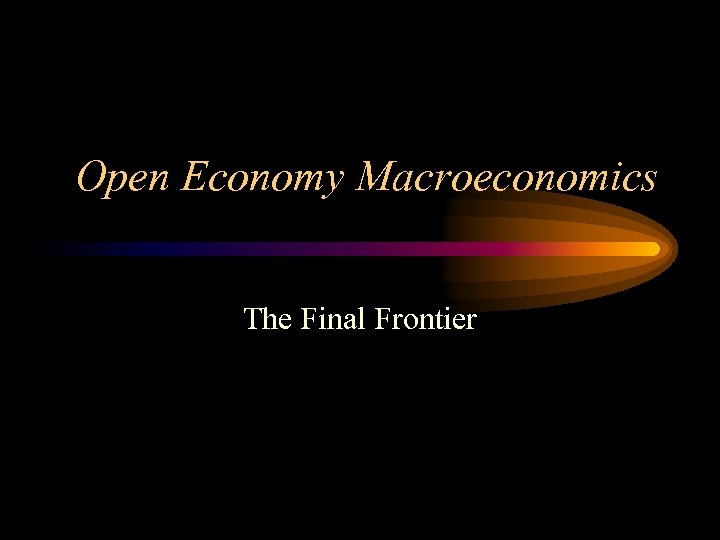Open Economy Macroeconomics The Final Frontier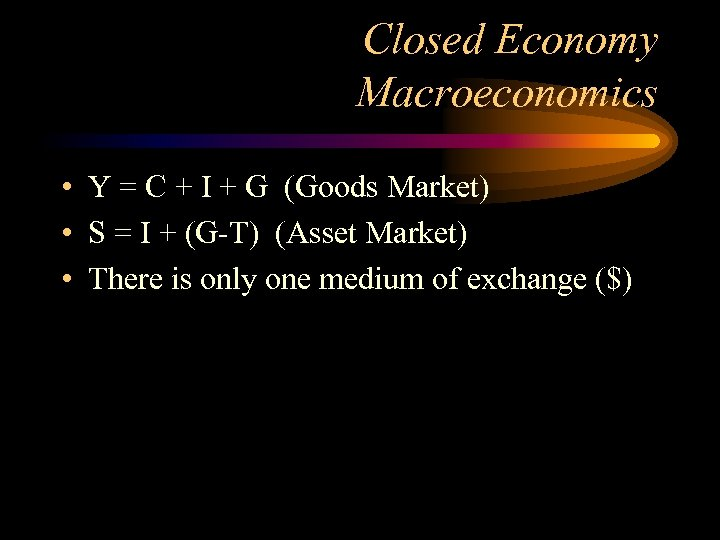Closed Economy Macroeconomics • Y = C + I + G (Goods Market) • S = I + (G-T) (Asset Market) • There is only one medium of exchange (\$)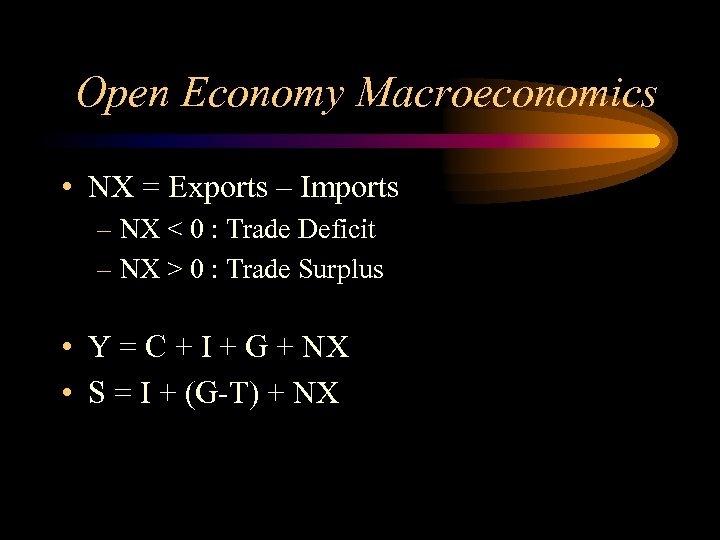Open Economy Macroeconomics • NX = Exports – Imports – NX < 0 : Trade Deficit – NX > 0 : Trade Surplus • Y = C + I + G + NX • S = I + (G-T) + NX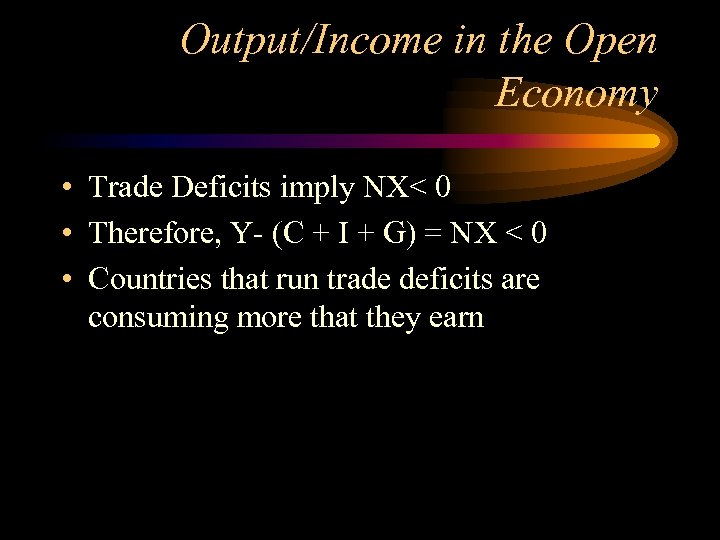Output/Income in the Open Economy • Trade Deficits imply NX< 0 • Therefore, Y- (C + I + G) = NX < 0 • Countries that run trade deficits are consuming more that they earn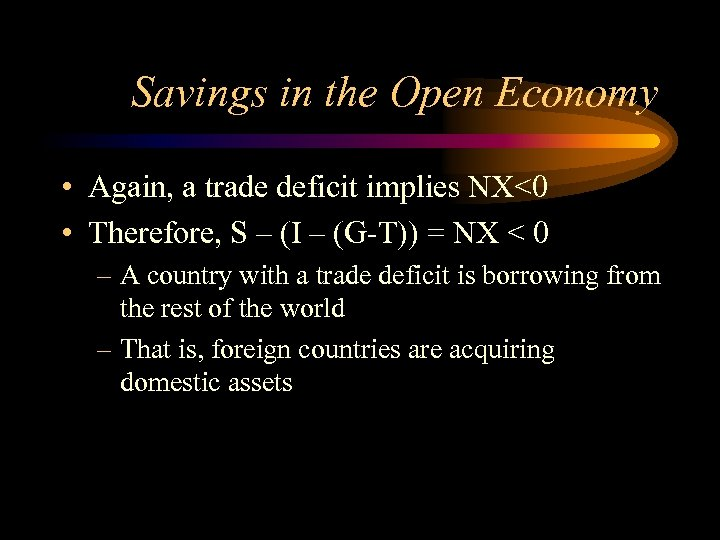Savings in the Open Economy • Again, a trade deficit implies NX<0 • Therefore, S – (I – (G-T)) = NX < 0 – A country with a trade deficit is borrowing from the rest of the world – That is, foreign countries are acquiring domestic assets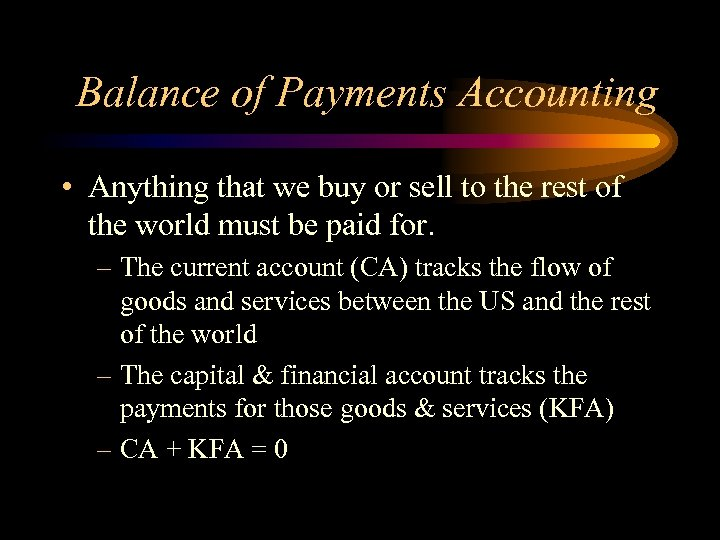Balance of Payments Accounting • Anything that we buy or sell to the rest of the world must be paid for. – The current account (CA) tracks the flow of goods and services between the US and the rest of the world – The capital & financial account tracks the payments for those goods & services (KFA) – CA + KFA = 0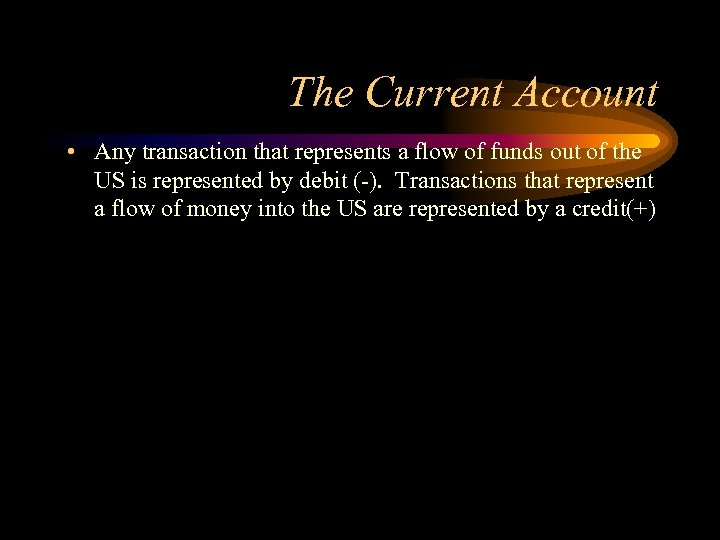The Current Account • Any transaction that represents a flow of funds out of the US is represented by debit (-). Transactions that represent a flow of money into the US are represented by a credit(+)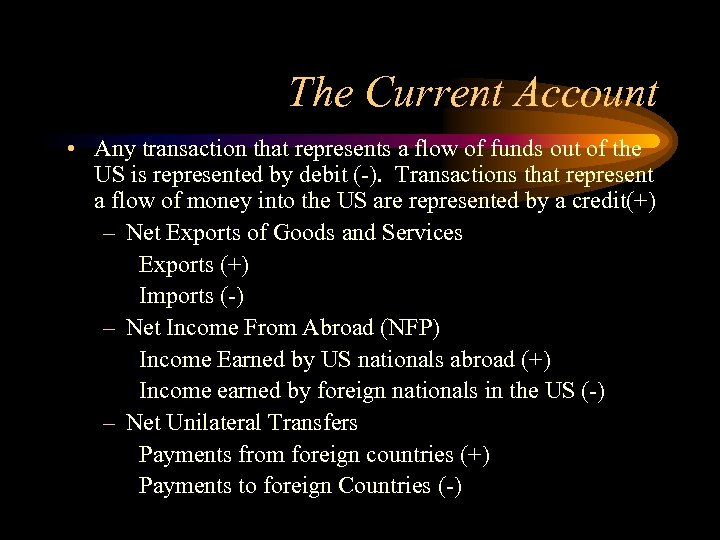The Current Account • Any transaction that represents a flow of funds out of the US is represented by debit (-). Transactions that represent a flow of money into the US are represented by a credit(+) – Net Exports of Goods and Services Exports (+) Imports (-) – Net Income From Abroad (NFP) Income Earned by US nationals abroad (+) Income earned by foreign nationals in the US (-) – Net Unilateral Transfers Payments from foreign countries (+) Payments to foreign Countries (-)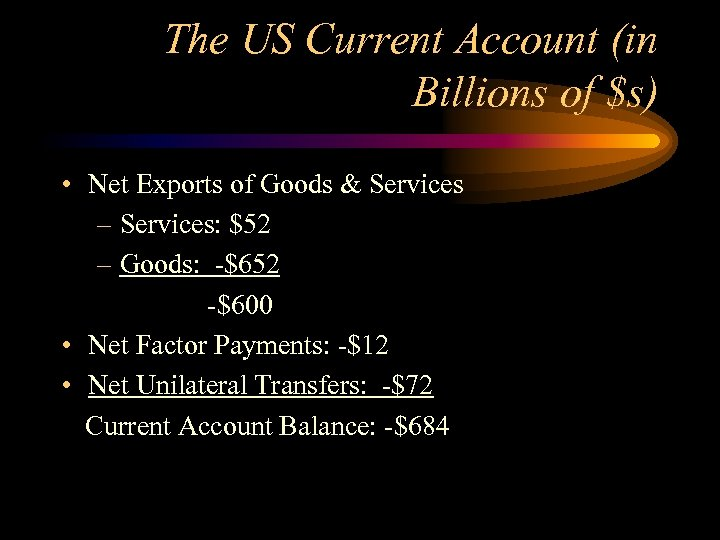The US Current Account (in Billions of \$s) • Net Exports of Goods & Services – Services: \$52 – Goods: -\$652 -\$600 • Net Factor Payments: -\$12 • Net Unilateral Transfers: -\$72 Current Account Balance: -\$684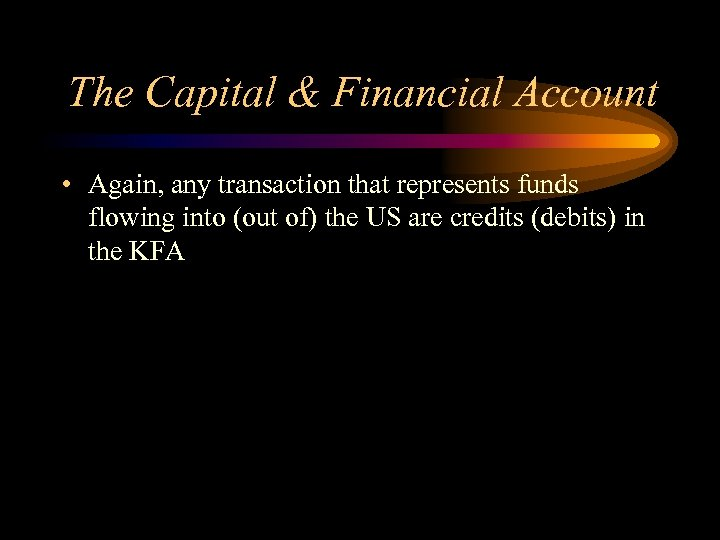The Capital & Financial Account • Again, any transaction that represents funds flowing into (out of) the US are credits (debits) in the KFA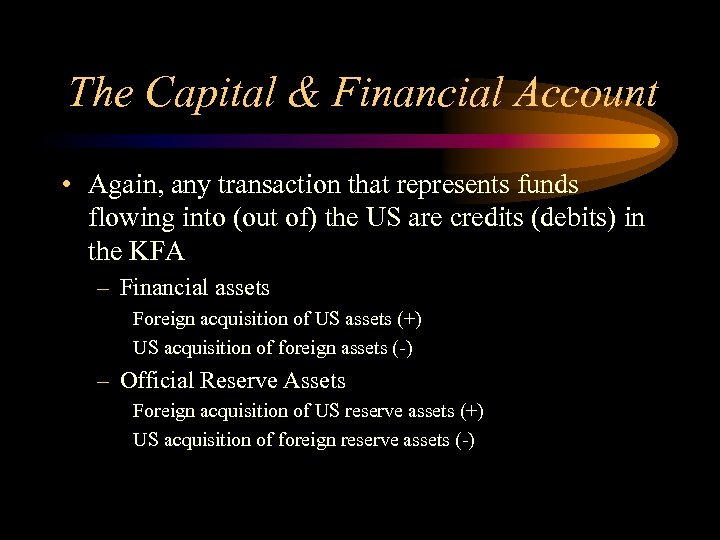The Capital & Financial Account • Again, any transaction that represents funds flowing into (out of) the US are credits (debits) in the KFA – Financial assets Foreign acquisition of US assets (+) US acquisition of foreign assets (-) – Official Reserve Assets Foreign acquisition of US reserve assets (+) US acquisition of foreign reserve assets (-)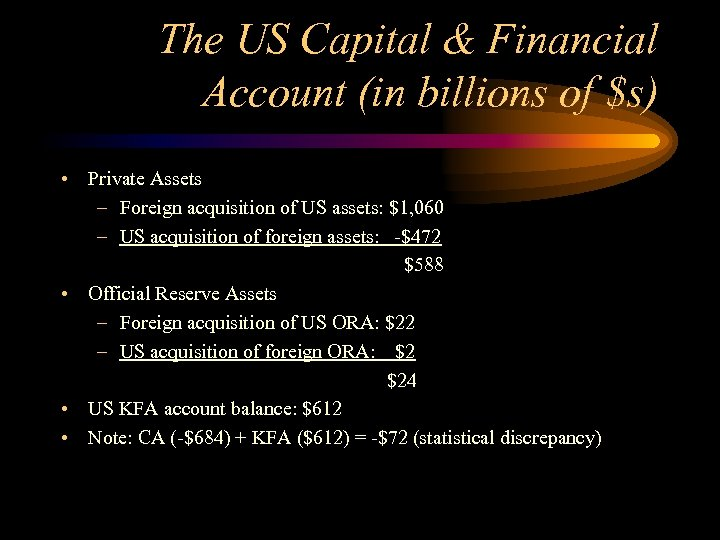The US Capital & Financial Account (in billions of \$s) • Private Assets – Foreign acquisition of US assets: \$1, 060 – US acquisition of foreign assets: -\$472 \$588 • Official Reserve Assets – Foreign acquisition of US ORA: \$22 – US acquisition of foreign ORA: \$2 \$24 • US KFA account balance: \$612 • Note: CA (-\$684) + KFA (\$612) = -\$72 (statistical discrepancy)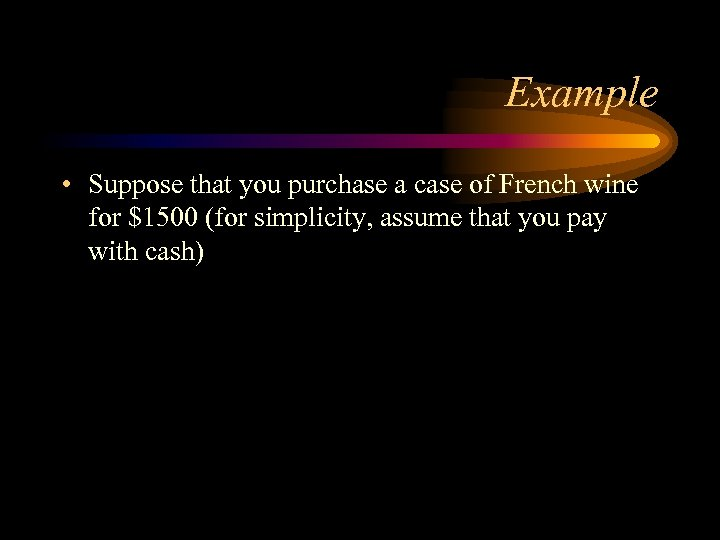Example • Suppose that you purchase a case of French wine for \$1500 (for simplicity, assume that you pay with cash)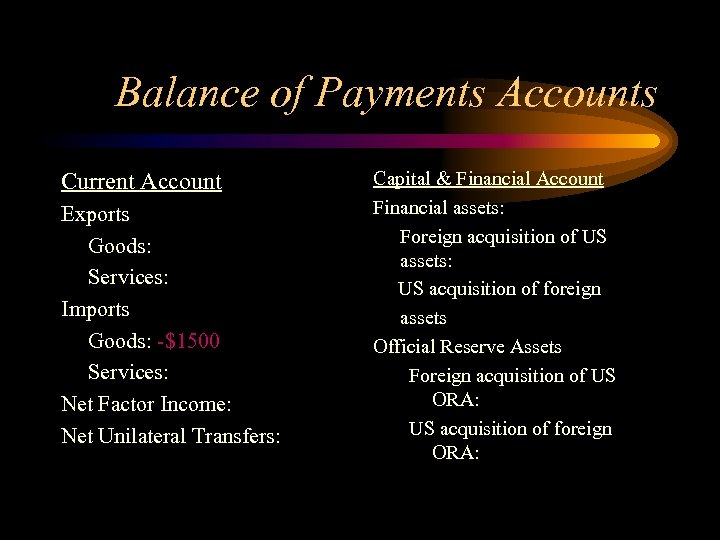Balance of Payments Accounts Current Account Exports Goods: Services: Imports Goods: -\$1500 Services: Net Factor Income: Net Unilateral Transfers: Capital & Financial Account Financial assets: Foreign acquisition of US assets: US acquisition of foreign assets Official Reserve Assets Foreign acquisition of US ORA: US acquisition of foreign ORA: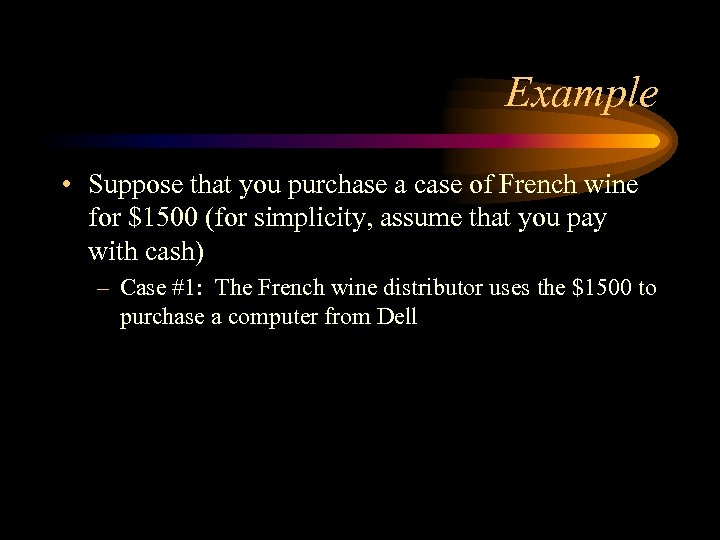Example • Suppose that you purchase a case of French wine for \$1500 (for simplicity, assume that you pay with cash) – Case #1: The French wine distributor uses the \$1500 to purchase a computer from Dell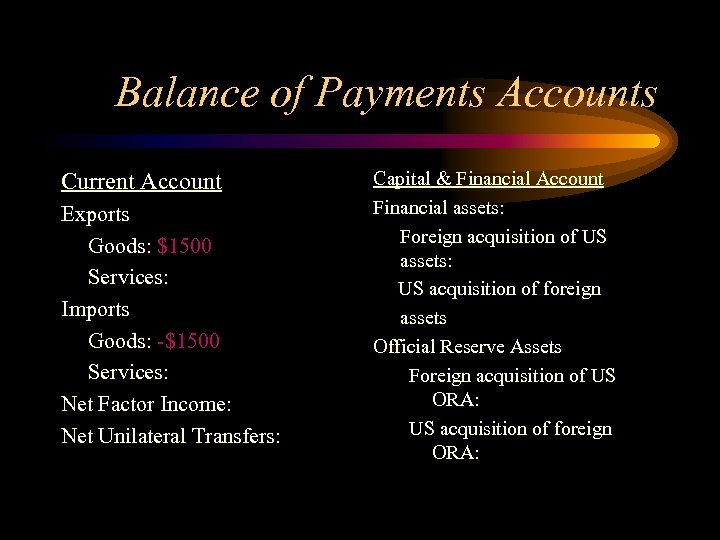Balance of Payments Accounts Current Account Exports Goods: \$1500 Services: Imports Goods: -\$1500 Services: Net Factor Income: Net Unilateral Transfers: Capital & Financial Account Financial assets: Foreign acquisition of US assets: US acquisition of foreign assets Official Reserve Assets Foreign acquisition of US ORA: US acquisition of foreign ORA: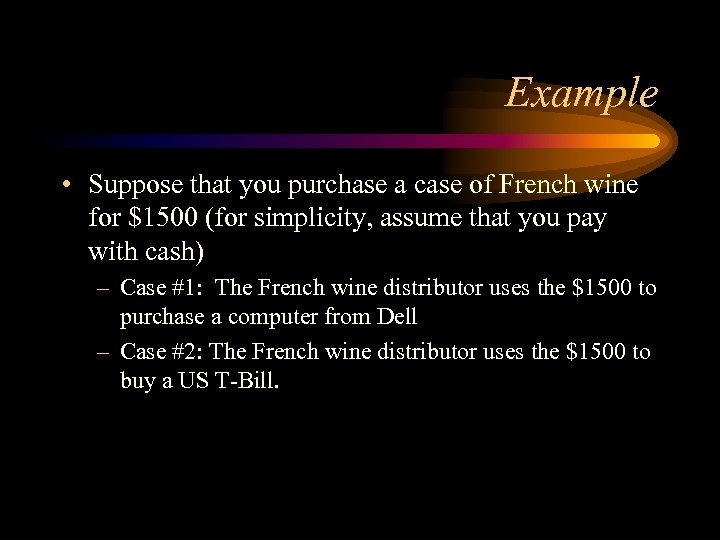Example • Suppose that you purchase a case of French wine for \$1500 (for simplicity, assume that you pay with cash) – Case #1: The French wine distributor uses the \$1500 to purchase a computer from Dell – Case #2: The French wine distributor uses the \$1500 to buy a US T-Bill.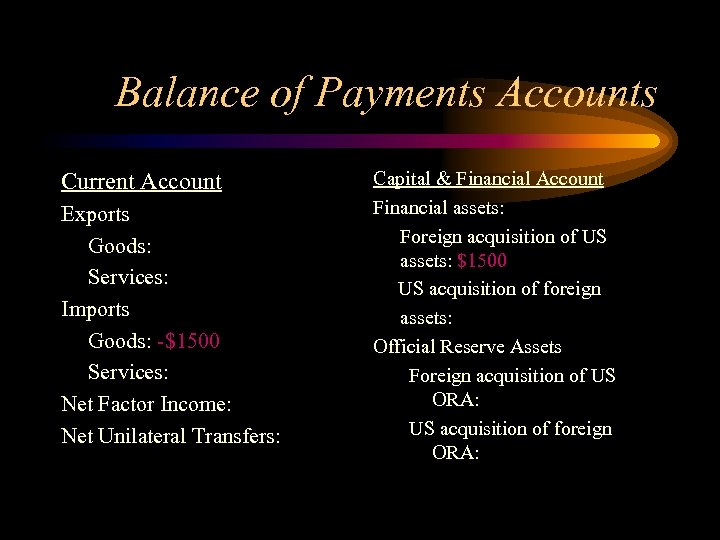Balance of Payments Accounts Current Account Exports Goods: Services: Imports Goods: -\$1500 Services: Net Factor Income: Net Unilateral Transfers: Capital & Financial Account Financial assets: Foreign acquisition of US assets: \$1500 US acquisition of foreign assets: Official Reserve Assets Foreign acquisition of US ORA: US acquisition of foreign ORA: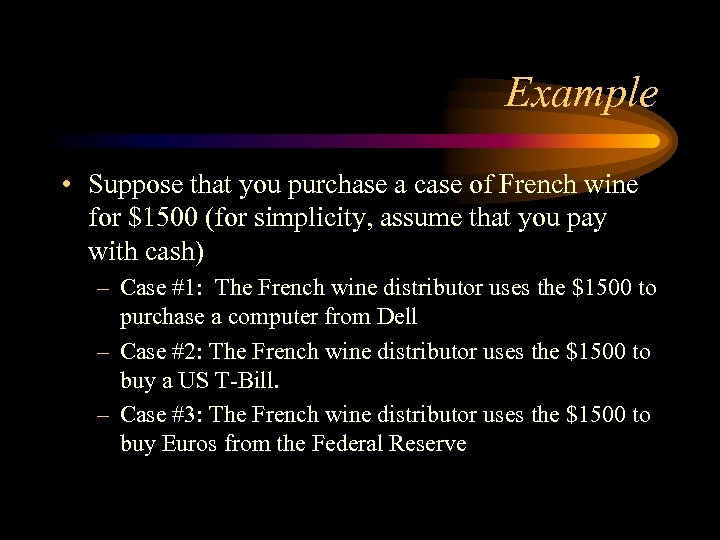Example • Suppose that you purchase a case of French wine for \$1500 (for simplicity, assume that you pay with cash) – Case #1: The French wine distributor uses the \$1500 to purchase a computer from Dell – Case #2: The French wine distributor uses the \$1500 to buy a US T-Bill. – Case #3: The French wine distributor uses the \$1500 to buy Euros from the Federal Reserve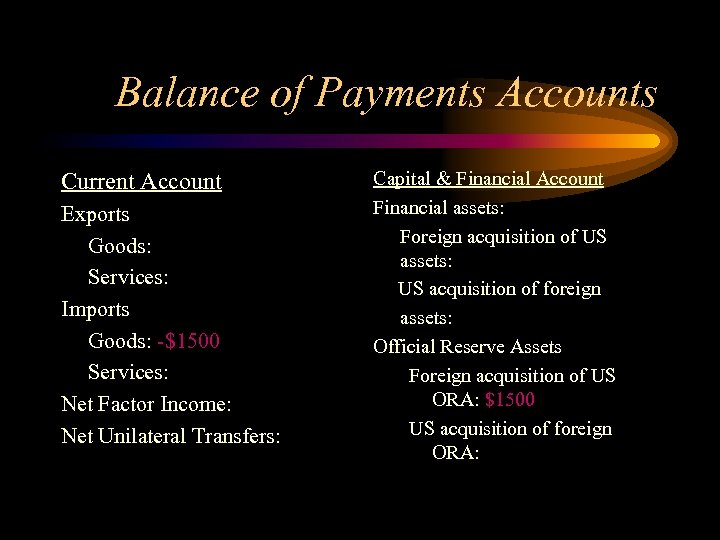Balance of Payments Accounts Current Account Exports Goods: Services: Imports Goods: -\$1500 Services: Net Factor Income: Net Unilateral Transfers: Capital & Financial Account Financial assets: Foreign acquisition of US assets: US acquisition of foreign assets: Official Reserve Assets Foreign acquisition of US ORA: \$1500 US acquisition of foreign ORA: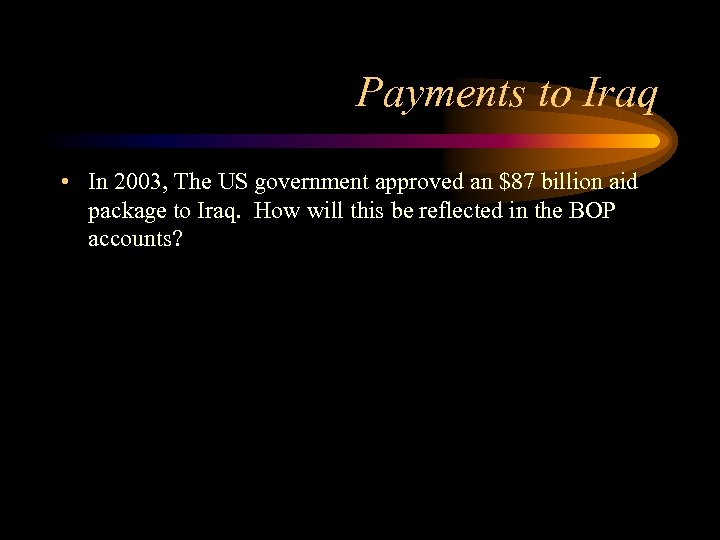Payments to Iraq • In 2003, The US government approved an \$87 billion aid package to Iraq. How will this be reflected in the BOP accounts?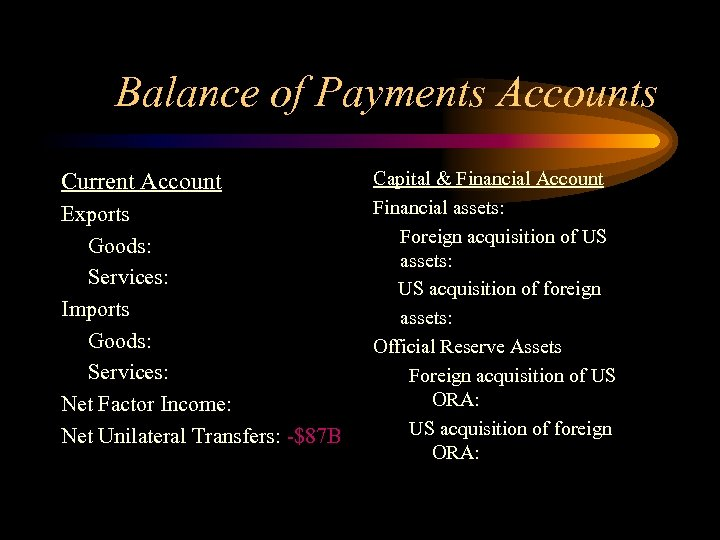Balance of Payments Accounts Current Account Exports Goods: Services: Imports Goods: Services: Net Factor Income: Net Unilateral Transfers: -\$87 B Capital & Financial Account Financial assets: Foreign acquisition of US assets: US acquisition of foreign assets: Official Reserve Assets Foreign acquisition of US ORA: US acquisition of foreign ORA: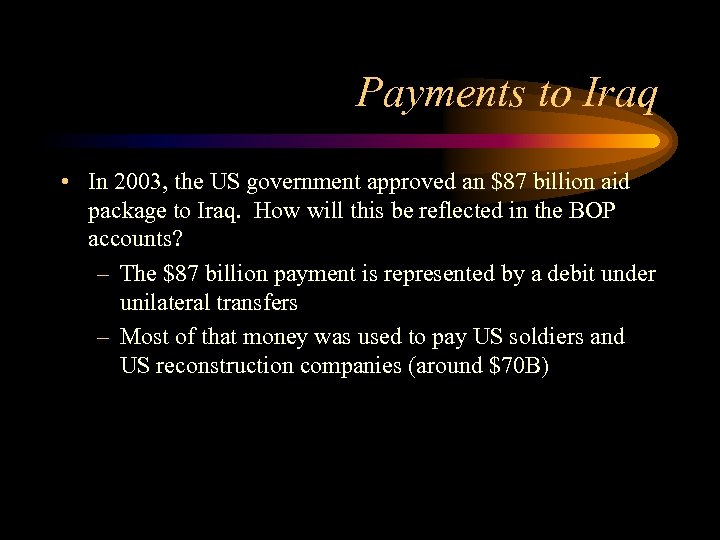Payments to Iraq • In 2003, the US government approved an \$87 billion aid package to Iraq. How will this be reflected in the BOP accounts? – The \$87 billion payment is represented by a debit under unilateral transfers – Most of that money was used to pay US soldiers and US reconstruction companies (around \$70 B)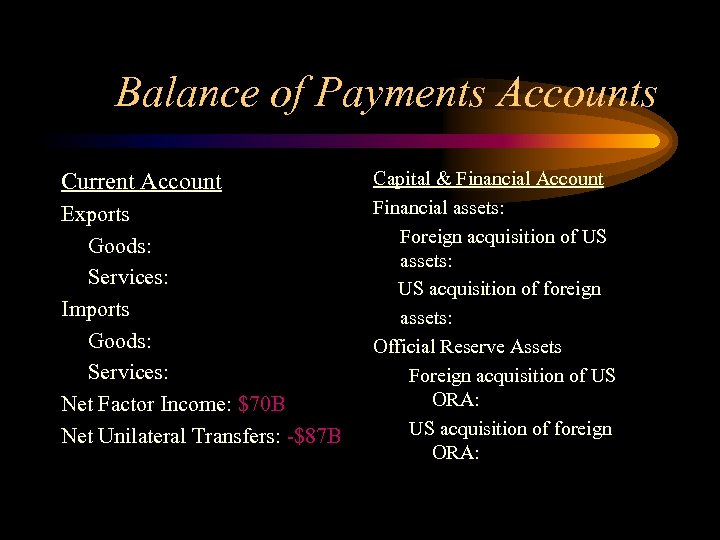Balance of Payments Accounts Current Account Exports Goods: Services: Imports Goods: Services: Net Factor Income: \$70 B Net Unilateral Transfers: -\$87 B Capital & Financial Account Financial assets: Foreign acquisition of US assets: US acquisition of foreign assets: Official Reserve Assets Foreign acquisition of US ORA: US acquisition of foreign ORA: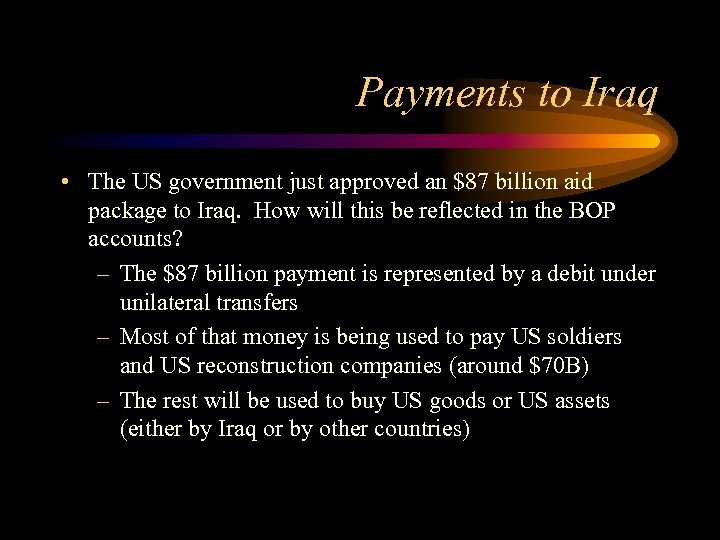Payments to Iraq • The US government just approved an \$87 billion aid package to Iraq. How will this be reflected in the BOP accounts? – The \$87 billion payment is represented by a debit under unilateral transfers – Most of that money is being used to pay US soldiers and US reconstruction companies (around \$70 B) – The rest will be used to buy US goods or US assets (either by Iraq or by other countries)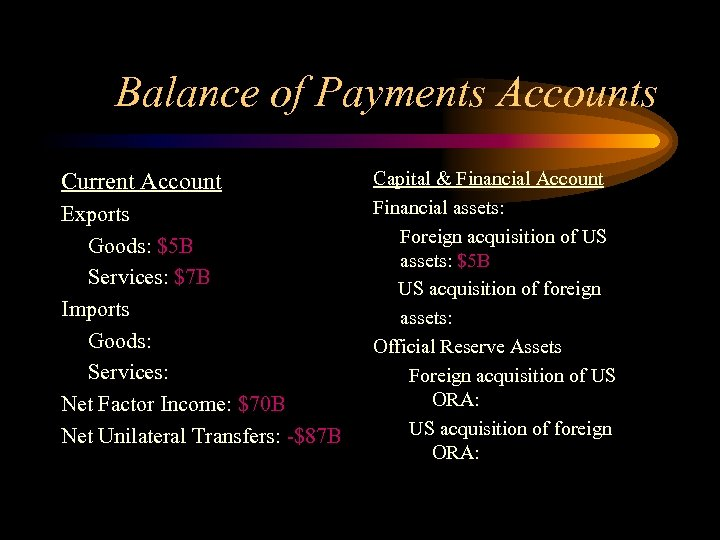Balance of Payments Accounts Current Account Exports Goods: \$5 B Services: \$7 B Imports Goods: Services: Net Factor Income: \$70 B Net Unilateral Transfers: -\$87 B Capital & Financial Account Financial assets: Foreign acquisition of US assets: \$5 B US acquisition of foreign assets: Official Reserve Assets Foreign acquisition of US ORA: US acquisition of foreign ORA: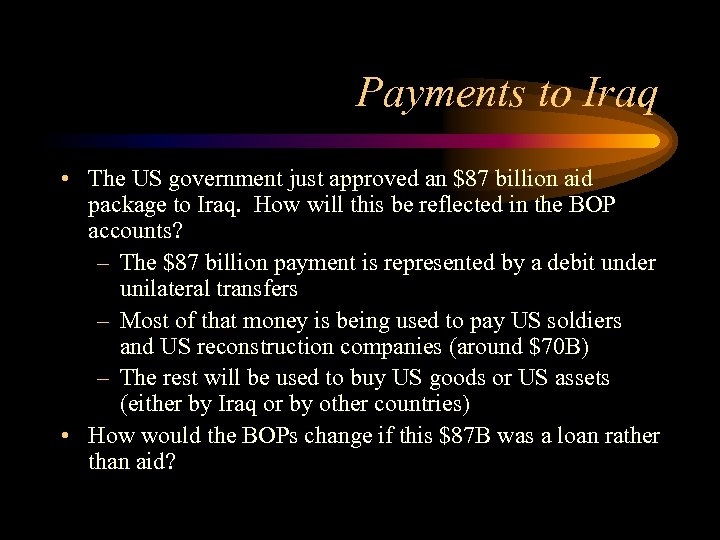Payments to Iraq • The US government just approved an \$87 billion aid package to Iraq. How will this be reflected in the BOP accounts? – The \$87 billion payment is represented by a debit under unilateral transfers – Most of that money is being used to pay US soldiers and US reconstruction companies (around \$70 B) – The rest will be used to buy US goods or US assets (either by Iraq or by other countries) • How would the BOPs change if this \$87 B was a loan rather than aid?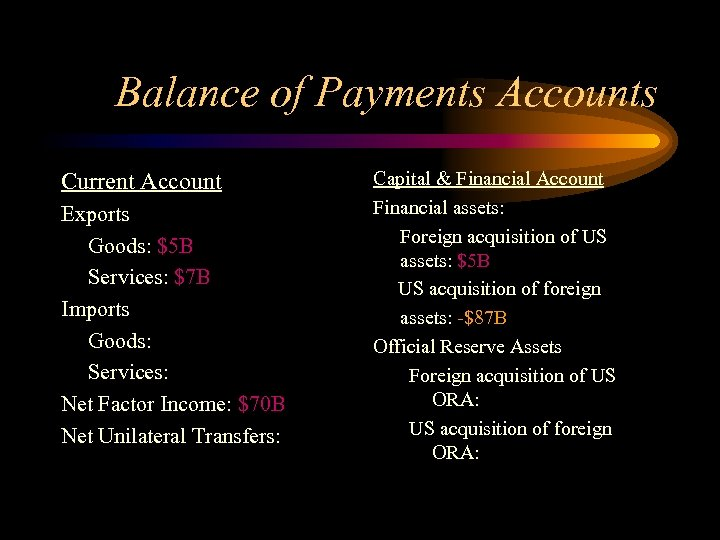Balance of Payments Accounts Current Account Exports Goods: \$5 B Services: \$7 B Imports Goods: Services: Net Factor Income: \$70 B Net Unilateral Transfers: Capital & Financial Account Financial assets: Foreign acquisition of US assets: \$5 B US acquisition of foreign assets: -\$87 B Official Reserve Assets Foreign acquisition of US ORA: US acquisition of foreign ORA: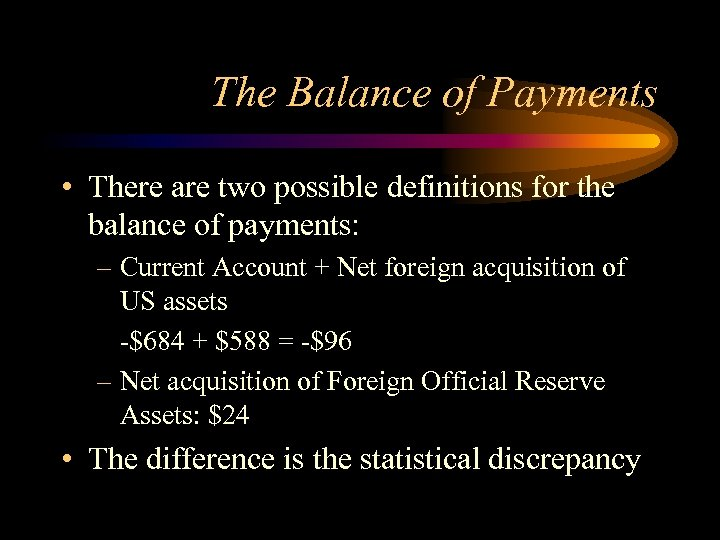The Balance of Payments • There are two possible definitions for the balance of payments: – Current Account + Net foreign acquisition of US assets -\$684 + \$588 = -\$96 – Net acquisition of Foreign Official Reserve Assets: \$24 • The difference is the statistical discrepancy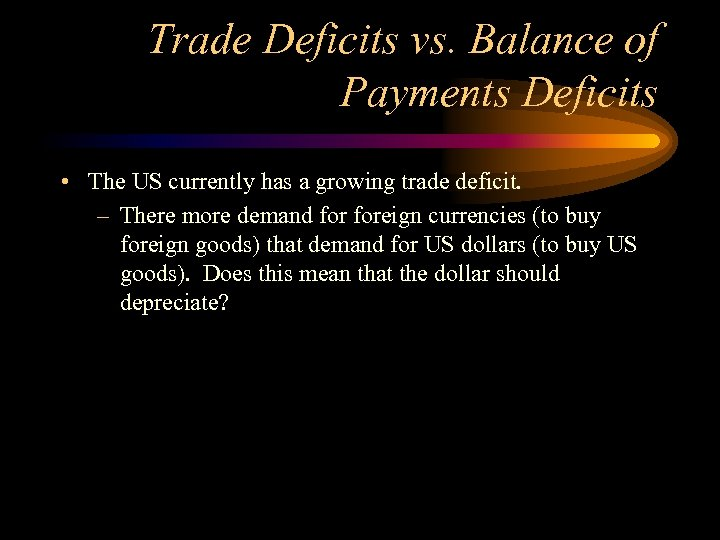Trade Deficits vs. Balance of Payments Deficits • The US currently has a growing trade deficit. – There more demand foreign currencies (to buy foreign goods) that demand for US dollars (to buy US goods). Does this mean that the dollar should depreciate?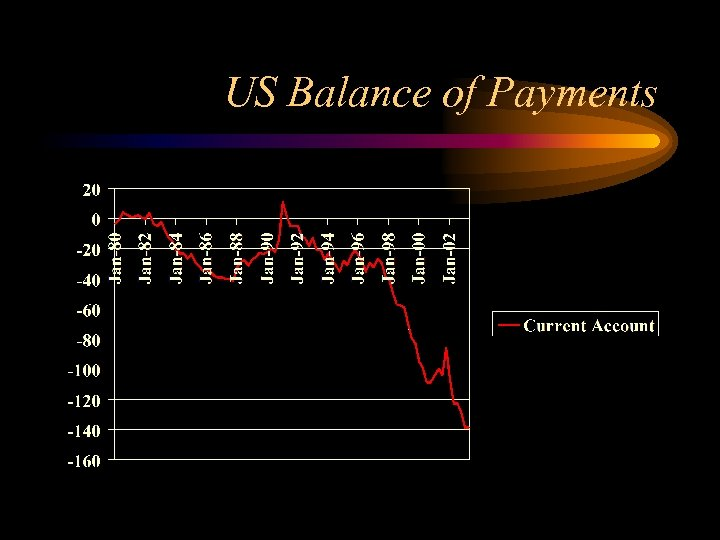US Balance of Payments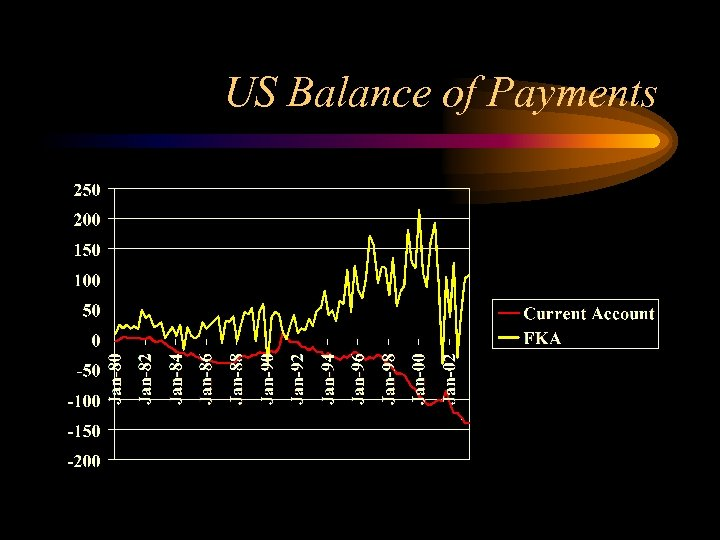US Balance of Payments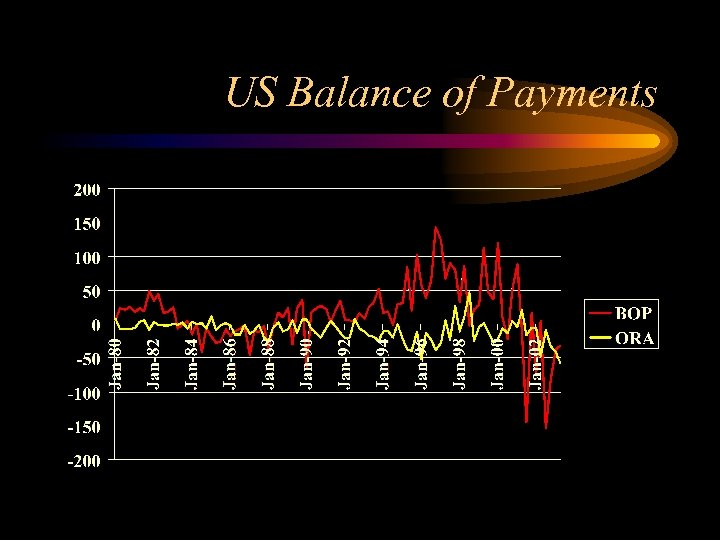US Balance of Payments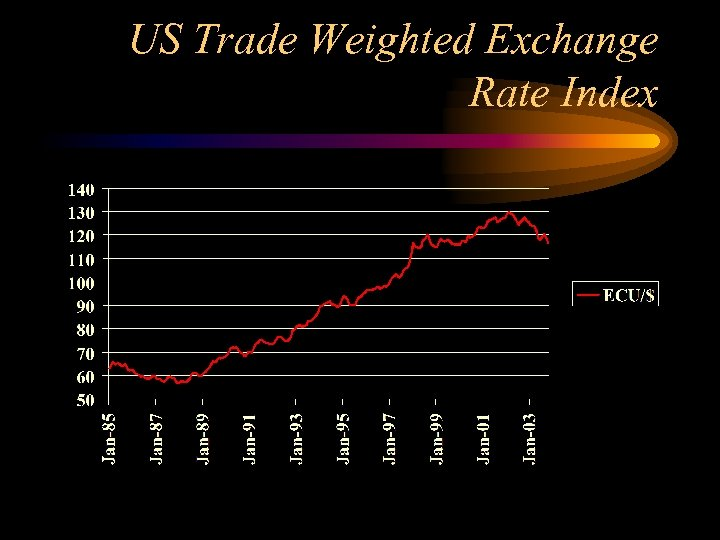US Trade Weighted Exchange Rate Index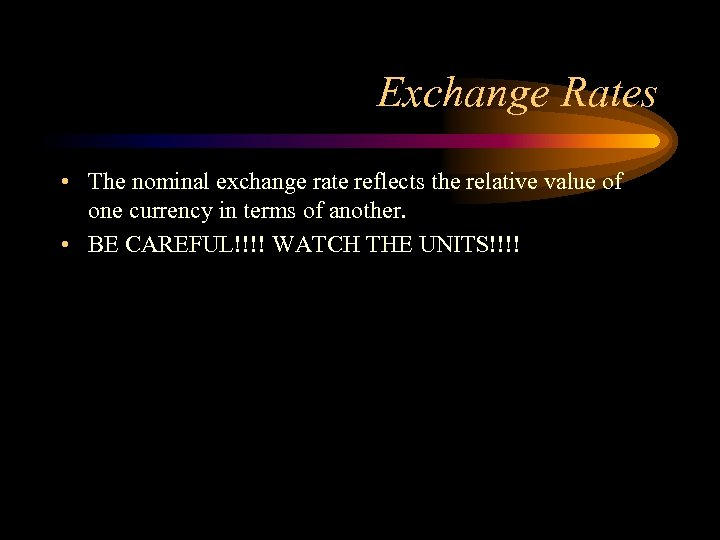Exchange Rates • The nominal exchange rate reflects the relative value of one currency in terms of another. • BE CAREFUL!!!! WATCH THE UNITS!!!!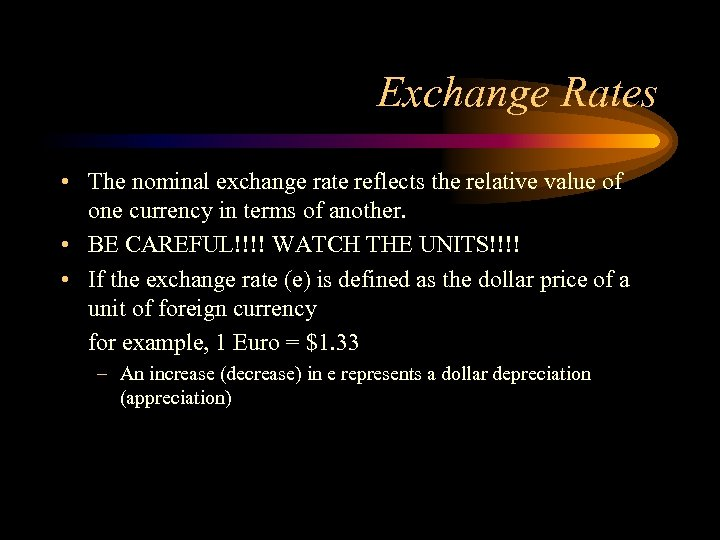Exchange Rates • The nominal exchange rate reflects the relative value of one currency in terms of another. • BE CAREFUL!!!! WATCH THE UNITS!!!! • If the exchange rate (e) is defined as the dollar price of a unit of foreign currency for example, 1 Euro = \$1. 33 – An increase (decrease) in e represents a dollar depreciation (appreciation)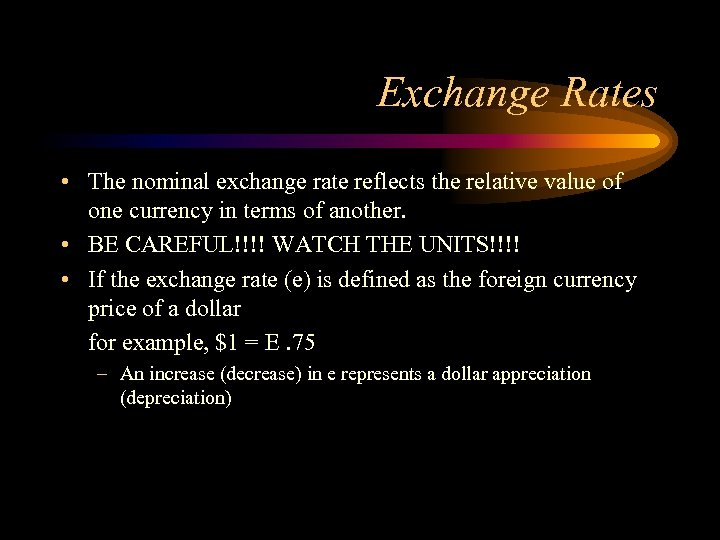Exchange Rates • The nominal exchange rate reflects the relative value of one currency in terms of another. • BE CAREFUL!!!! WATCH THE UNITS!!!! • If the exchange rate (e) is defined as the foreign currency price of a dollar for example, \$1 = E. 75 – An increase (decrease) in e represents a dollar appreciation (depreciation)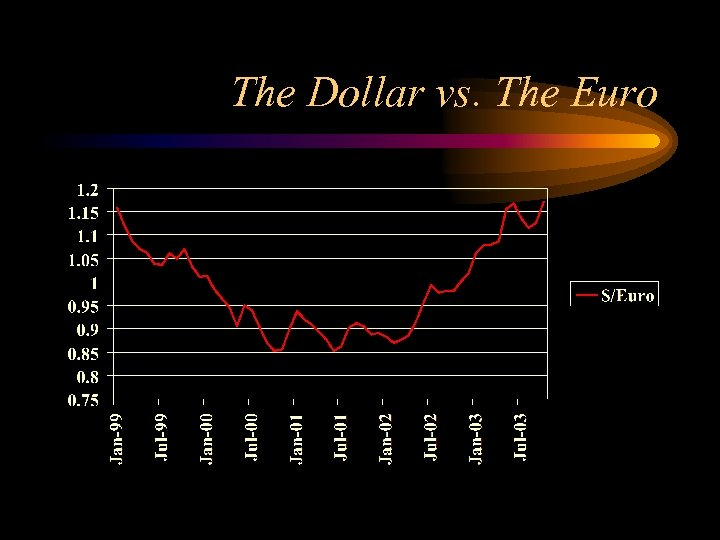The Dollar vs. The Euro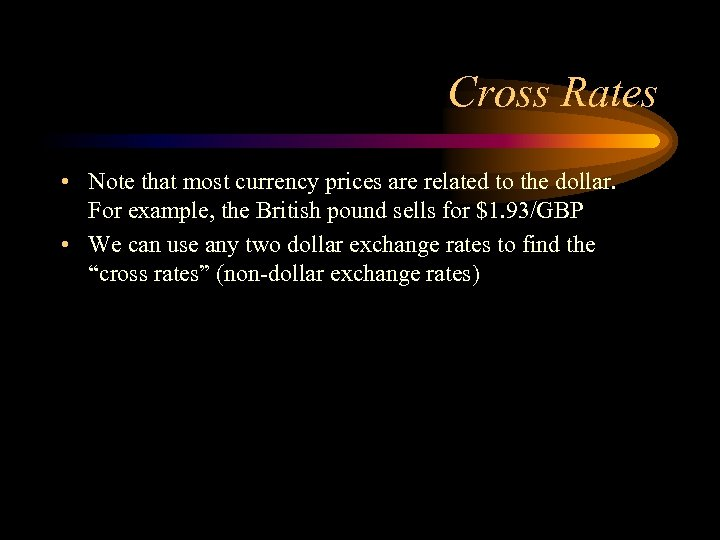Cross Rates • Note that most currency prices are related to the dollar. For example, the British pound sells for \$1. 93/GBP • We can use any two dollar exchange rates to find the “cross rates” (non-dollar exchange rates)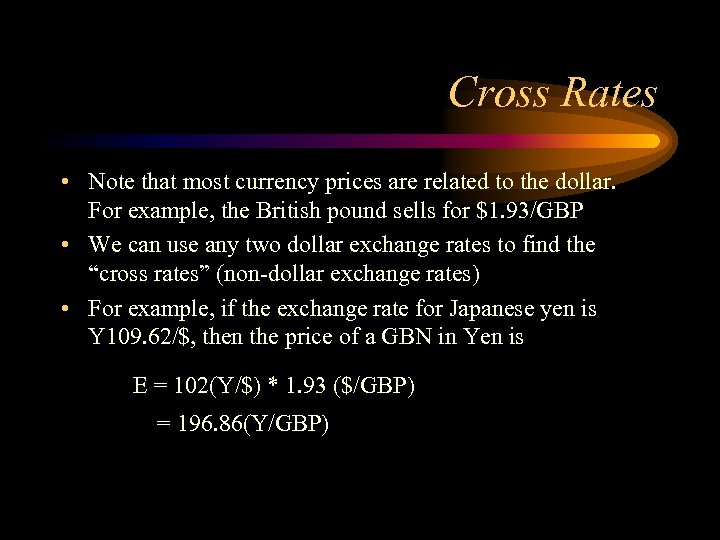Cross Rates • Note that most currency prices are related to the dollar. For example, the British pound sells for \$1. 93/GBP • We can use any two dollar exchange rates to find the “cross rates” (non-dollar exchange rates) • For example, if the exchange rate for Japanese yen is Y 109. 62/\$, then the price of a GBN in Yen is E = 102(Y/\$) * 1. 93 (\$/GBP) = 196. 86(Y/GBP)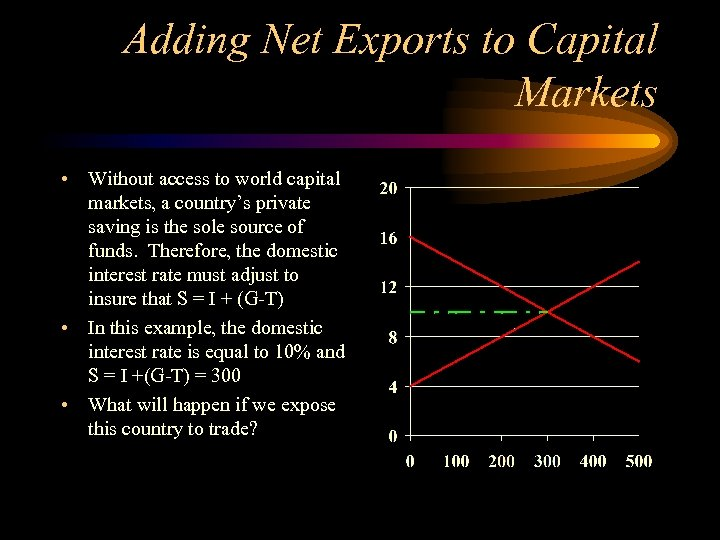Adding Net Exports to Capital Markets • Without access to world capital markets, a country’s private saving is the sole source of funds. Therefore, the domestic interest rate must adjust to insure that S = I + (G-T) • In this example, the domestic interest rate is equal to 10% and S = I +(G-T) = 300 • What will happen if we expose this country to trade?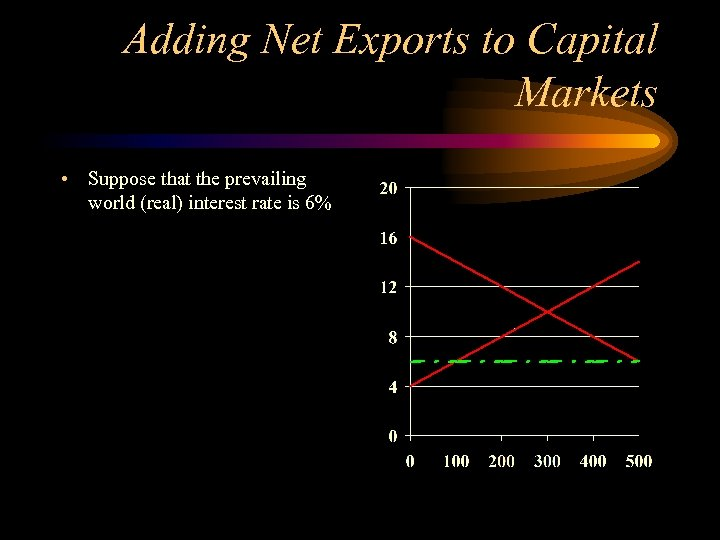Adding Net Exports to Capital Markets • Suppose that the prevailing world (real) interest rate is 6%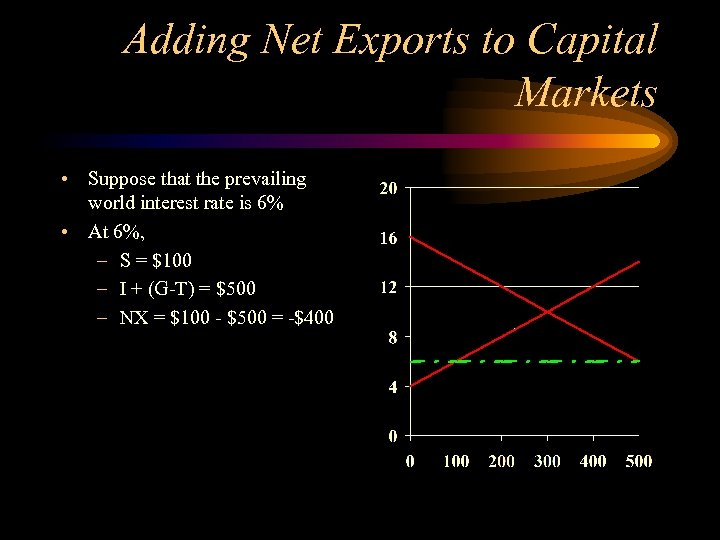Adding Net Exports to Capital Markets • Suppose that the prevailing world interest rate is 6% • At 6%, – S = \$100 – I + (G-T) = \$500 – NX = \$100 - \$500 = -\$400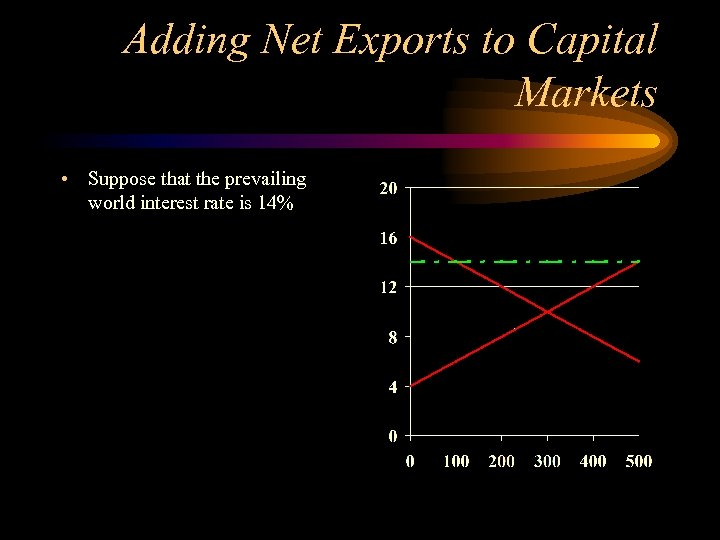Adding Net Exports to Capital Markets • Suppose that the prevailing world interest rate is 14%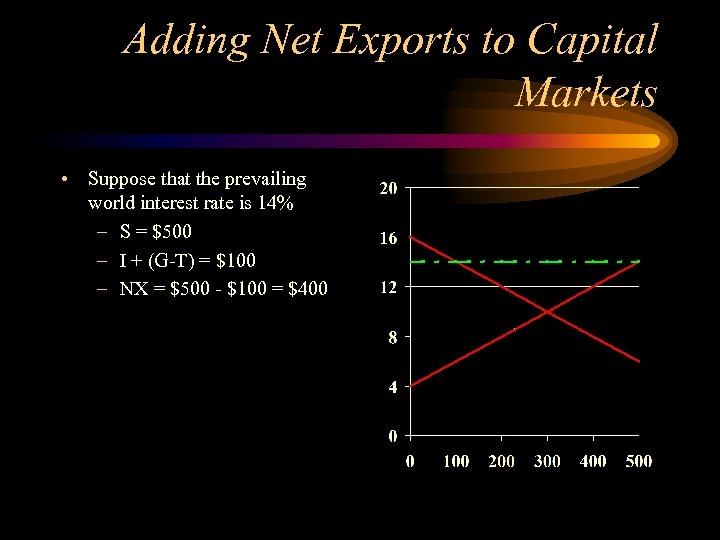Adding Net Exports to Capital Markets • Suppose that the prevailing world interest rate is 14% – S = \$500 – I + (G-T) = \$100 – NX = \$500 - \$100 = \$400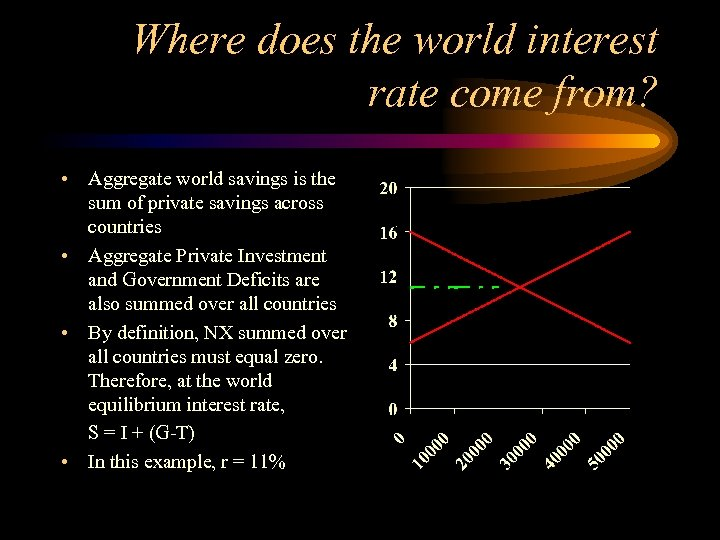Where does the world interest rate come from? • Aggregate world savings is the sum of private savings across countries • Aggregate Private Investment and Government Deficits are also summed over all countries • By definition, NX summed over all countries must equal zero. Therefore, at the world equilibrium interest rate, S = I + (G-T) • In this example, r = 11%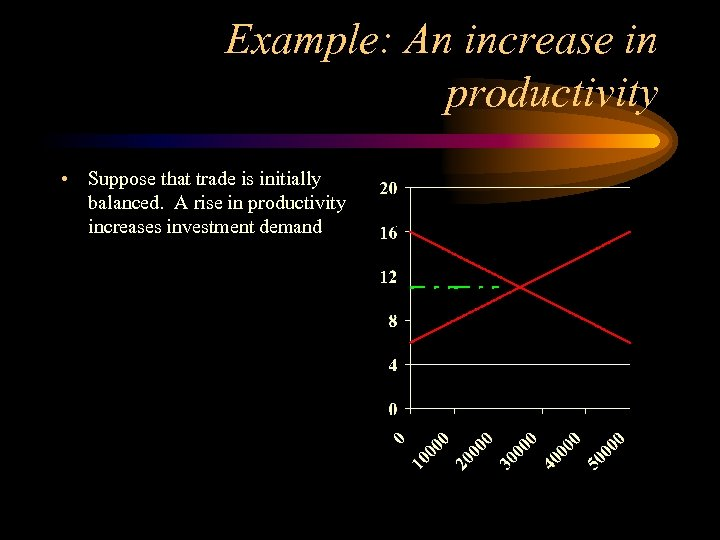Example: An increase in productivity • Suppose that trade is initially balanced. A rise in productivity increases investment demand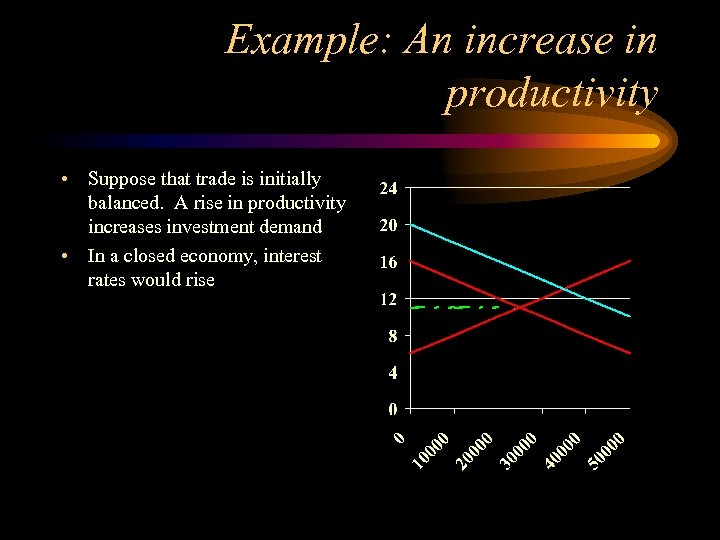Example: An increase in productivity • Suppose that trade is initially balanced. A rise in productivity increases investment demand • In a closed economy, interest rates would rise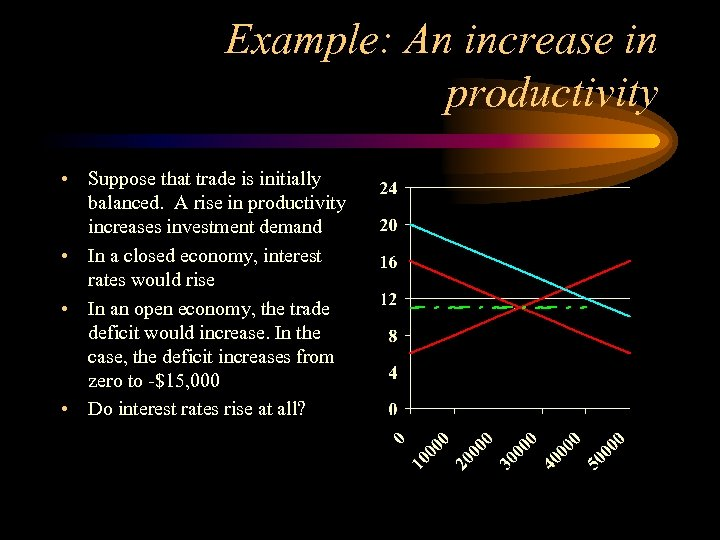Example: An increase in productivity • Suppose that trade is initially balanced. A rise in productivity increases investment demand • In a closed economy, interest rates would rise • In an open economy, the trade deficit would increase. In the case, the deficit increases from zero to -\$15, 000 • Do interest rates rise at all?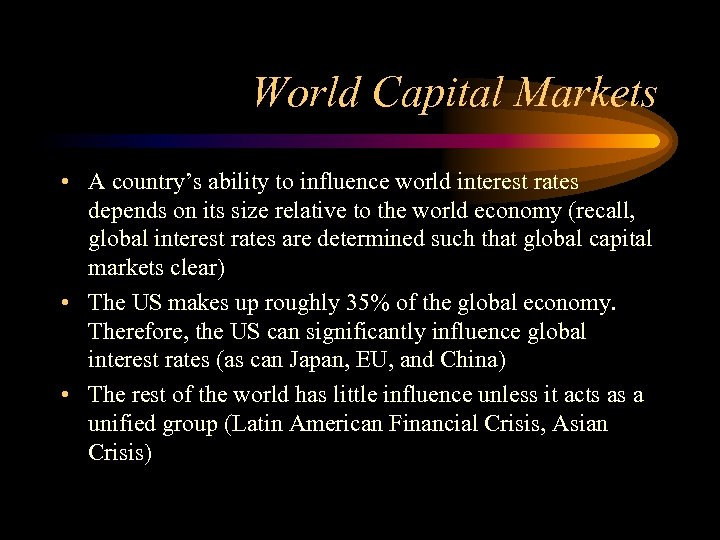World Capital Markets • A country’s ability to influence world interest rates depends on its size relative to the world economy (recall, global interest rates are determined such that global capital markets clear) • The US makes up roughly 35% of the global economy. Therefore, the US can significantly influence global interest rates (as can Japan, EU, and China) • The rest of the world has little influence unless it acts as a unified group (Latin American Financial Crisis, Asian Crisis)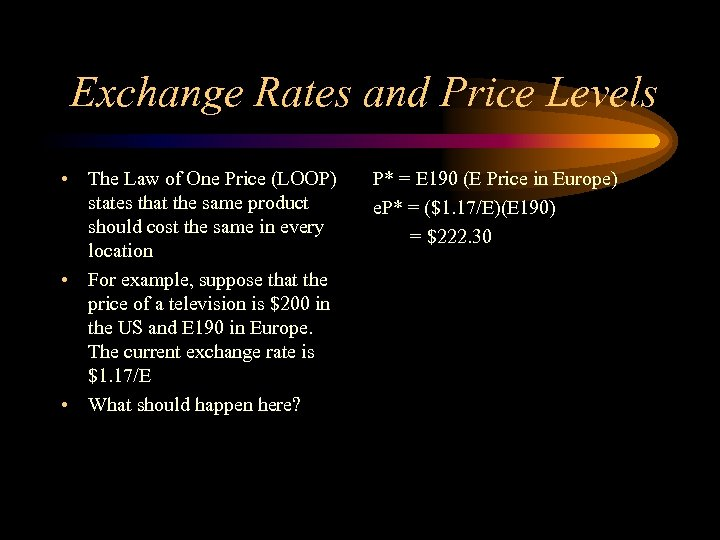Exchange Rates and Price Levels • The Law of One Price (LOOP) states that the same product should cost the same in every location • For example, suppose that the price of a television is \$200 in the US and E 190 in Europe. The current exchange rate is \$1. 17/E • What should happen here? P* = E 190 (E Price in Europe) e. P* = (\$1. 17/E)(E 190) = \$222. 30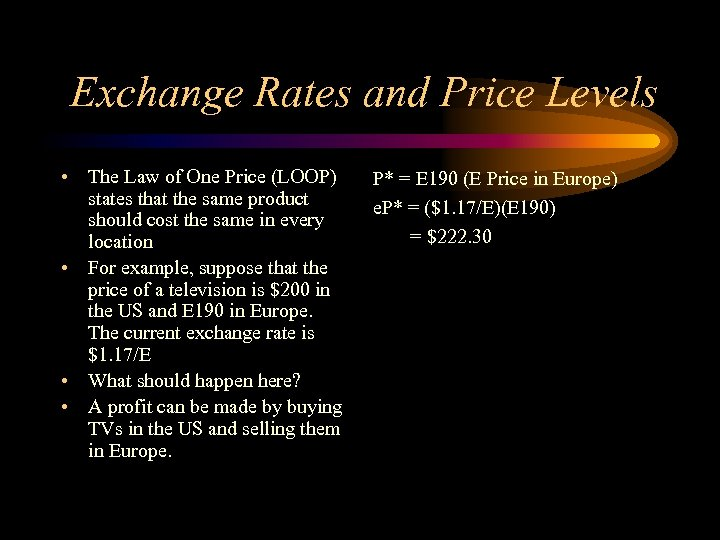Exchange Rates and Price Levels • The Law of One Price (LOOP) states that the same product should cost the same in every location • For example, suppose that the price of a television is \$200 in the US and E 190 in Europe. The current exchange rate is \$1. 17/E • What should happen here? • A profit can be made by buying TVs in the US and selling them in Europe. P* = E 190 (E Price in Europe) e. P* = (\$1. 17/E)(E 190) = \$222. 30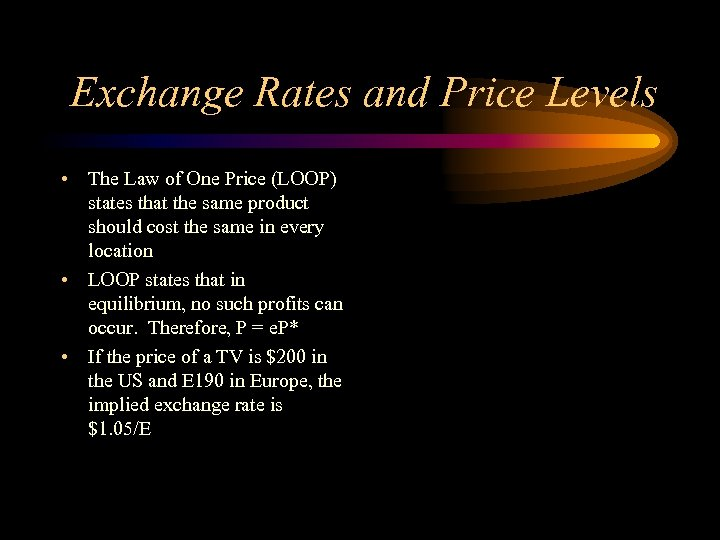Exchange Rates and Price Levels • The Law of One Price (LOOP) states that the same product should cost the same in every location • LOOP states that in equilibrium, no such profits can occur. Therefore, P = e. P* • If the price of a TV is \$200 in the US and E 190 in Europe, the implied exchange rate is \$1. 05/E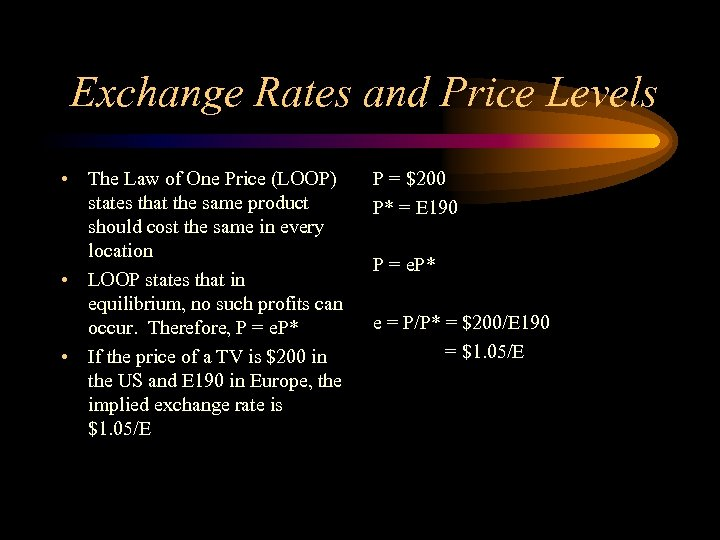Exchange Rates and Price Levels • The Law of One Price (LOOP) states that the same product should cost the same in every location • LOOP states that in equilibrium, no such profits can occur. Therefore, P = e. P* • If the price of a TV is \$200 in the US and E 190 in Europe, the implied exchange rate is \$1. 05/E P = \$200 P* = E 190 P = e. P* e = P/P* = \$200/E 190 = \$1. 05/E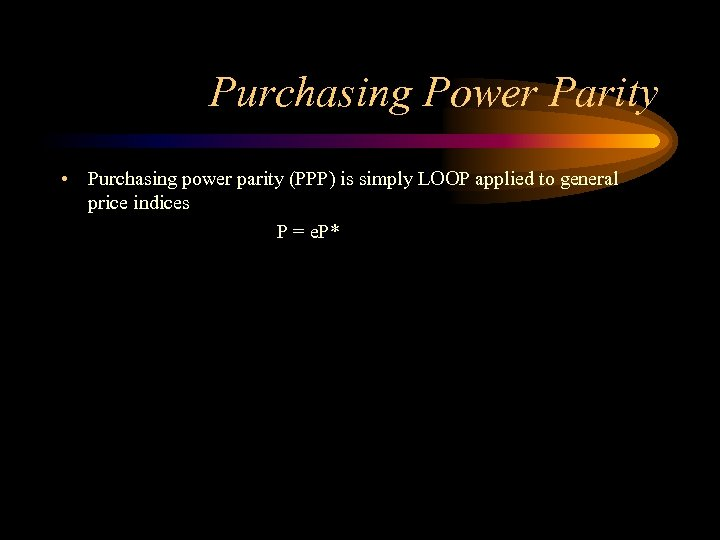Purchasing Power Parity • Purchasing power parity (PPP) is simply LOOP applied to general price indices P = e. P*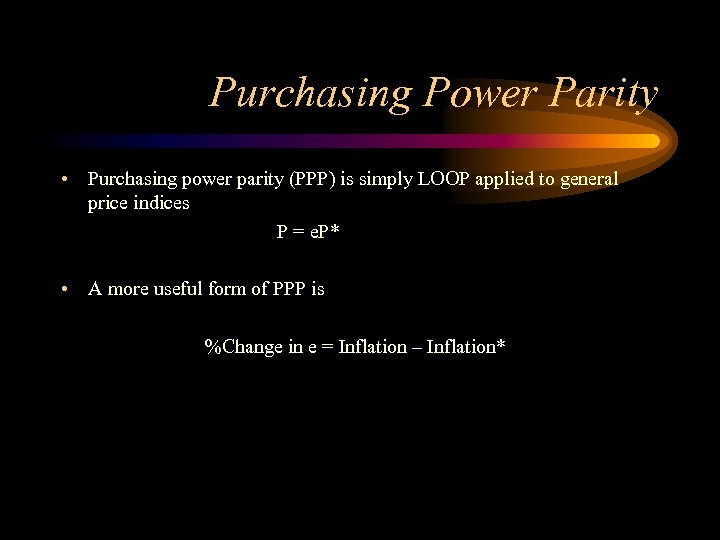Purchasing Power Parity • Purchasing power parity (PPP) is simply LOOP applied to general price indices P = e. P* • A more useful form of PPP is %Change in e = Inflation – Inflation*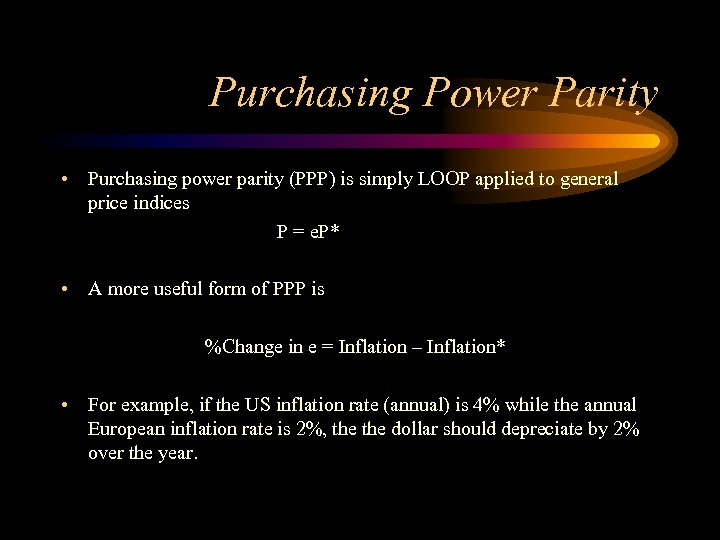Purchasing Power Parity • Purchasing power parity (PPP) is simply LOOP applied to general price indices P = e. P* • A more useful form of PPP is %Change in e = Inflation – Inflation* • For example, if the US inflation rate (annual) is 4% while the annual European inflation rate is 2%, the dollar should depreciate by 2% over the year.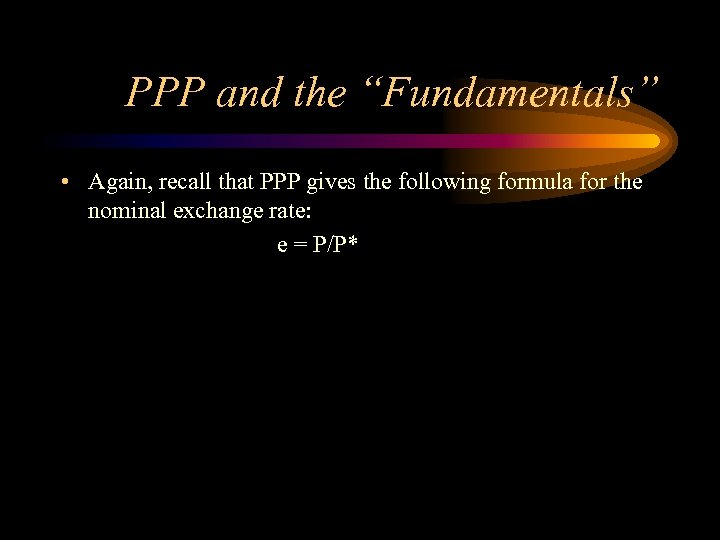PPP and the “Fundamentals” • Again, recall that PPP gives the following formula for the nominal exchange rate: e = P/P*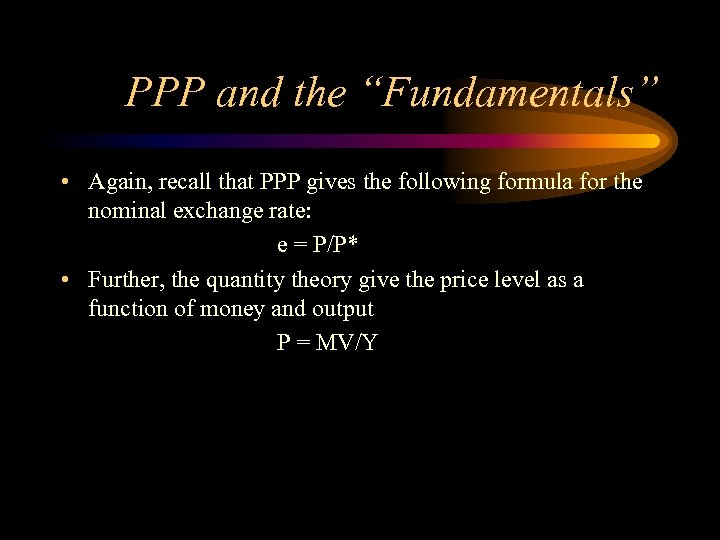PPP and the “Fundamentals” • Again, recall that PPP gives the following formula for the nominal exchange rate: e = P/P* • Further, the quantity theory give the price level as a function of money and output P = MV/Y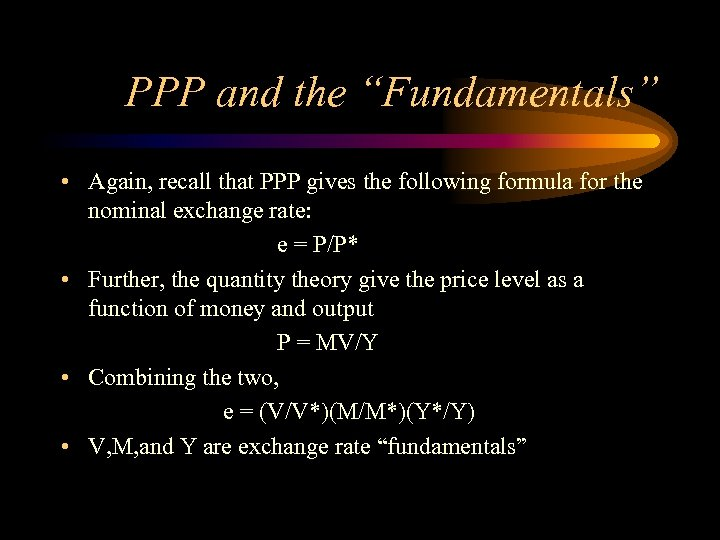PPP and the “Fundamentals” • Again, recall that PPP gives the following formula for the nominal exchange rate: e = P/P* • Further, the quantity theory give the price level as a function of money and output P = MV/Y • Combining the two, e = (V/V*)(M/M*)(Y*/Y) • V, M, and Y are exchange rate “fundamentals”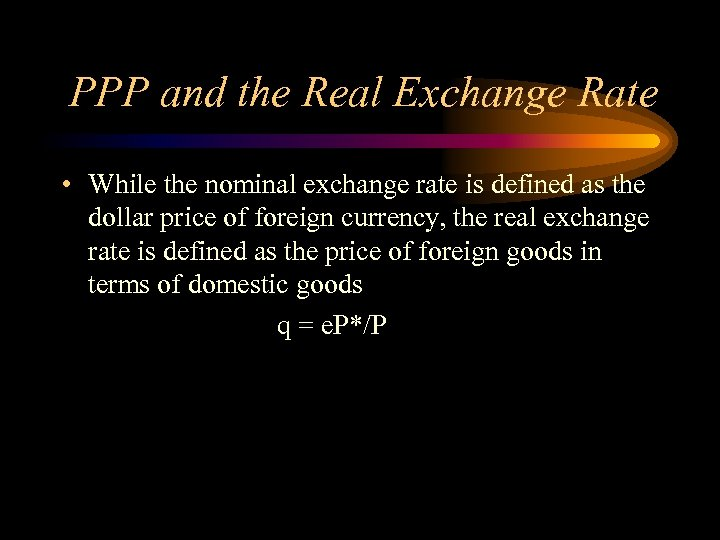PPP and the Real Exchange Rate • While the nominal exchange rate is defined as the dollar price of foreign currency, the real exchange rate is defined as the price of foreign goods in terms of domestic goods q = e. P*/P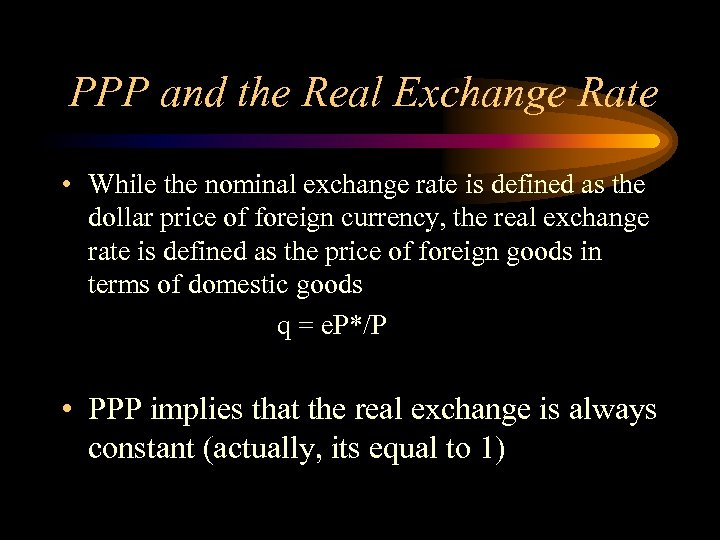PPP and the Real Exchange Rate • While the nominal exchange rate is defined as the dollar price of foreign currency, the real exchange rate is defined as the price of foreign goods in terms of domestic goods q = e. P*/P • PPP implies that the real exchange is always constant (actually, its equal to 1)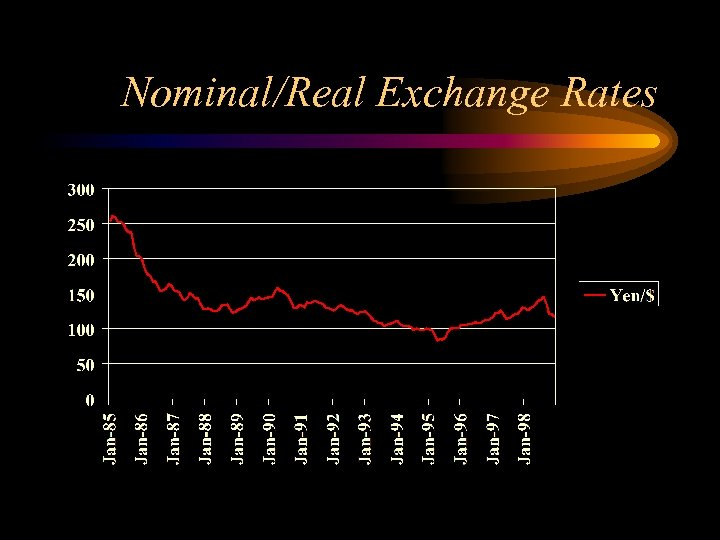Nominal/Real Exchange Rates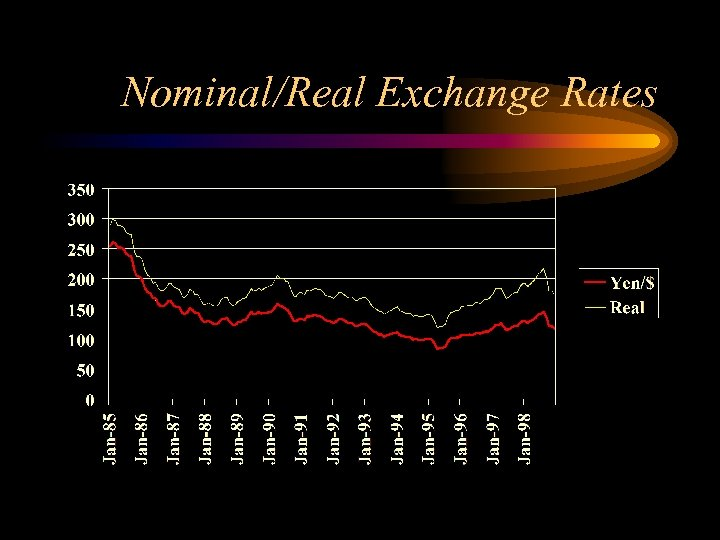Nominal/Real Exchange Rates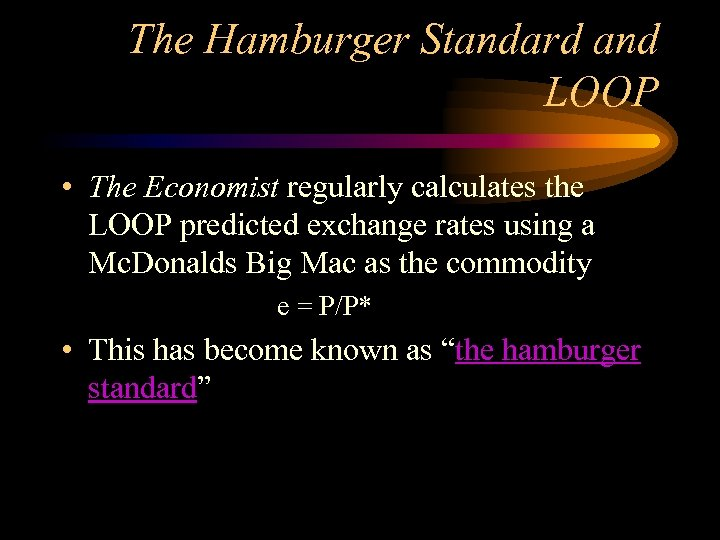The Hamburger Standard and LOOP • The Economist regularly calculates the LOOP predicted exchange rates using a Mc. Donalds Big Mac as the commodity e = P/P* • This has become known as “the hamburger standard”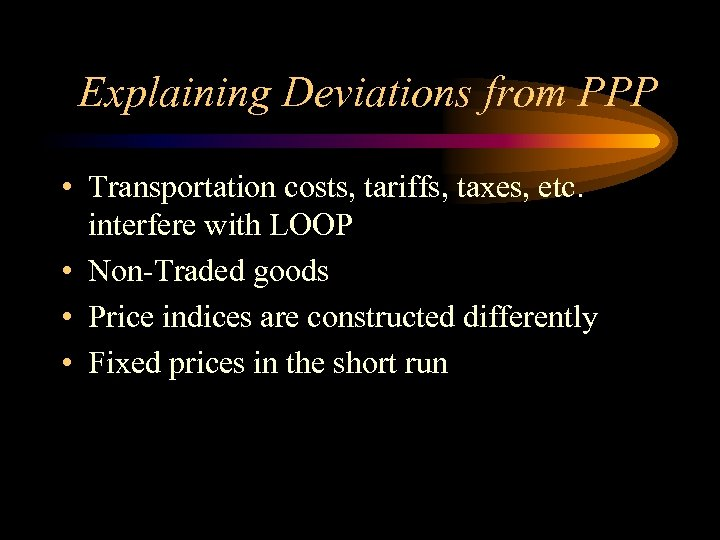Explaining Deviations from PPP • Transportation costs, tariffs, taxes, etc. interfere with LOOP • Non-Traded goods • Price indices are constructed differently • Fixed prices in the short run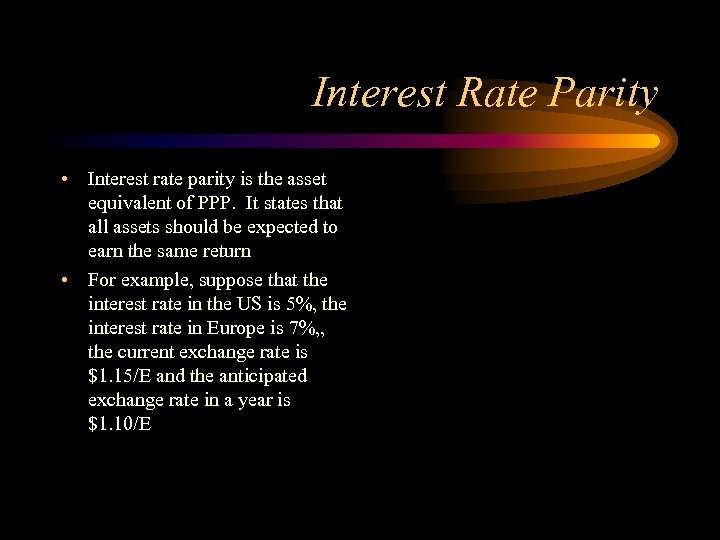Interest Rate Parity • Interest rate parity is the asset equivalent of PPP. It states that all assets should be expected to earn the same return • For example, suppose that the interest rate in the US is 5%, the interest rate in Europe is 7%, , the current exchange rate is \$1. 15/E and the anticipated exchange rate in a year is \$1. 10/E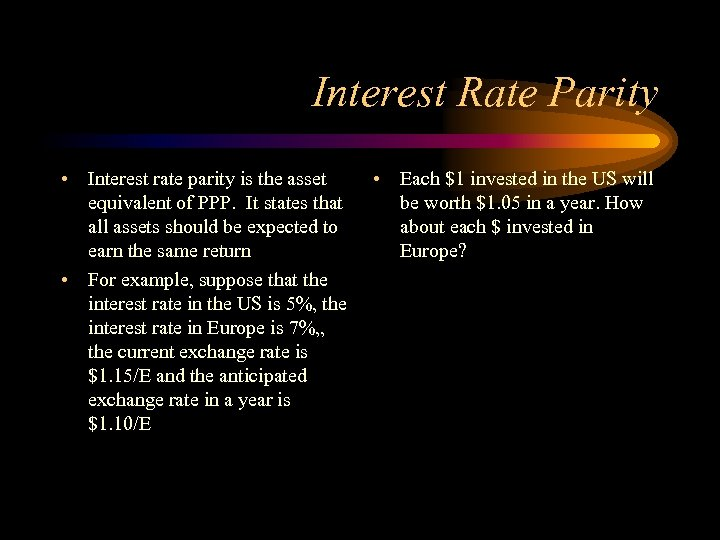Interest Rate Parity • Interest rate parity is the asset equivalent of PPP. It states that all assets should be expected to earn the same return • For example, suppose that the interest rate in the US is 5%, the interest rate in Europe is 7%, , the current exchange rate is \$1. 15/E and the anticipated exchange rate in a year is \$1. 10/E • Each \$1 invested in the US will be worth \$1. 05 in a year. How about each \$ invested in Europe?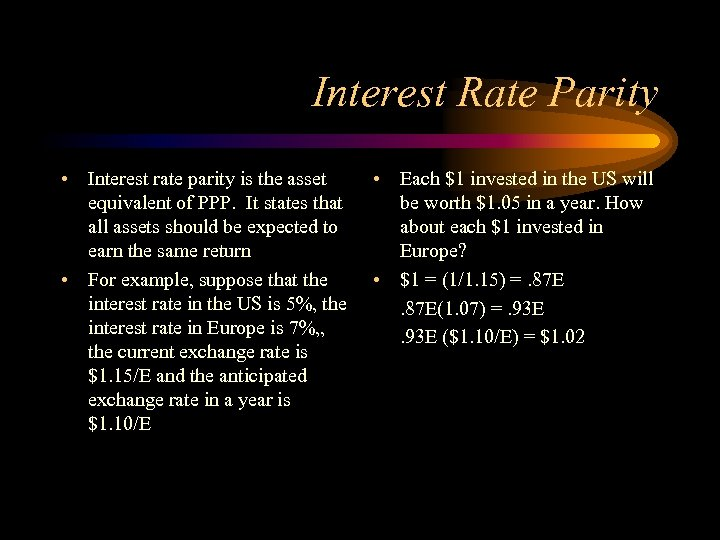Interest Rate Parity • Interest rate parity is the asset equivalent of PPP. It states that all assets should be expected to earn the same return • For example, suppose that the interest rate in the US is 5%, the interest rate in Europe is 7%, , the current exchange rate is \$1. 15/E and the anticipated exchange rate in a year is \$1. 10/E • Each \$1 invested in the US will be worth \$1. 05 in a year. How about each \$1 invested in Europe? • \$1 = (1/1. 15) =. 87 E(1. 07) =. 93 E (\$1. 10/E) = \$1. 02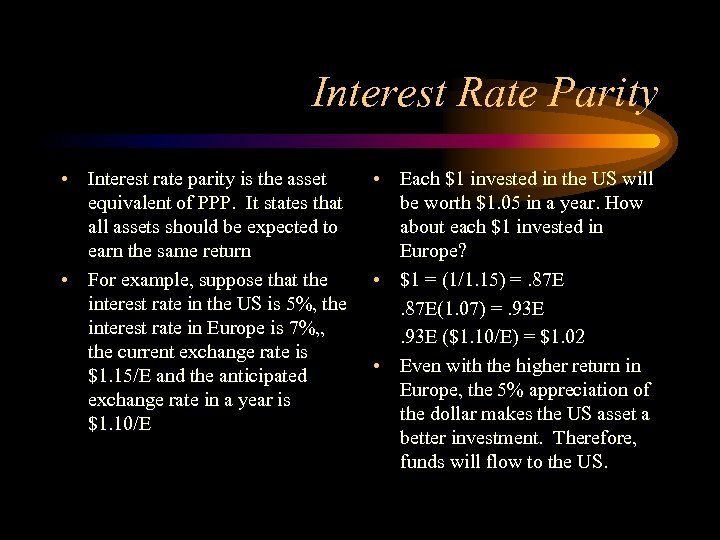Interest Rate Parity • Interest rate parity is the asset equivalent of PPP. It states that all assets should be expected to earn the same return • For example, suppose that the interest rate in the US is 5%, the interest rate in Europe is 7%, , the current exchange rate is \$1. 15/E and the anticipated exchange rate in a year is \$1. 10/E • Each \$1 invested in the US will be worth \$1. 05 in a year. How about each \$1 invested in Europe? • \$1 = (1/1. 15) =. 87 E(1. 07) =. 93 E (\$1. 10/E) = \$1. 02 • Even with the higher return in Europe, the 5% appreciation of the dollar makes the US asset a better investment. Therefore, funds will flow to the US.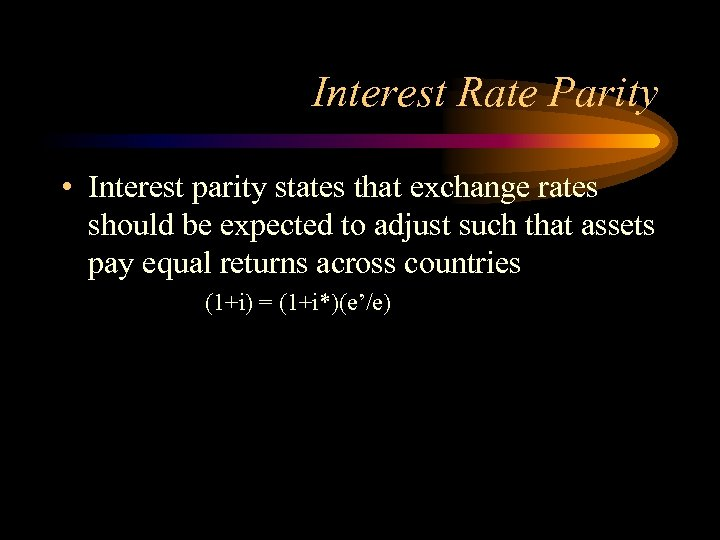Interest Rate Parity • Interest parity states that exchange rates should be expected to adjust such that assets pay equal returns across countries (1+i) = (1+i*)(e’/e)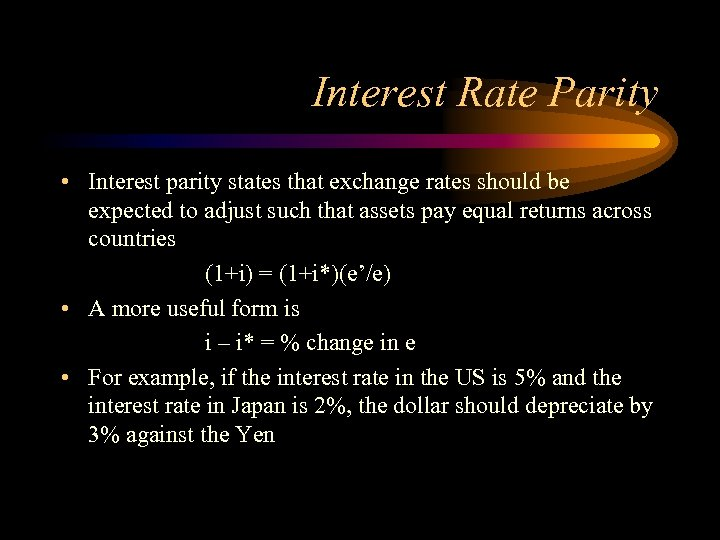Interest Rate Parity • Interest parity states that exchange rates should be expected to adjust such that assets pay equal returns across countries (1+i) = (1+i*)(e’/e) • A more useful form is i – i* = % change in e • For example, if the interest rate in the US is 5% and the interest rate in Japan is 2%, the dollar should depreciate by 3% against the Yen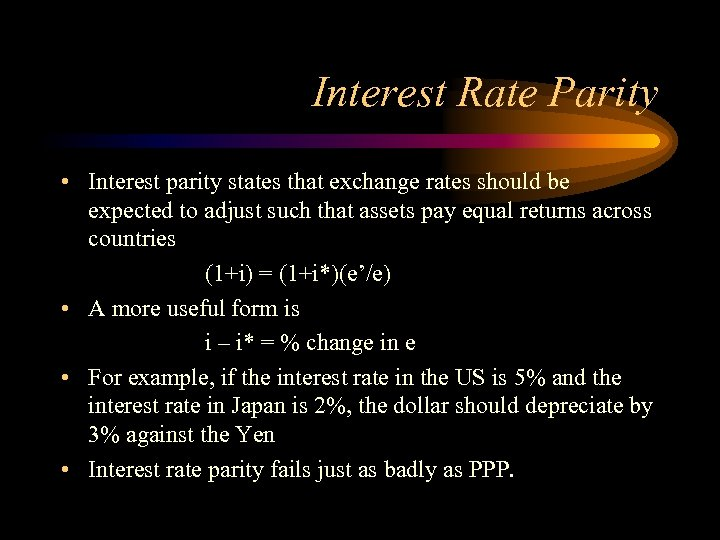Interest Rate Parity • Interest parity states that exchange rates should be expected to adjust such that assets pay equal returns across countries (1+i) = (1+i*)(e’/e) • A more useful form is i – i* = % change in e • For example, if the interest rate in the US is 5% and the interest rate in Japan is 2%, the dollar should depreciate by 3% against the Yen • Interest rate parity fails just as badly as PPP.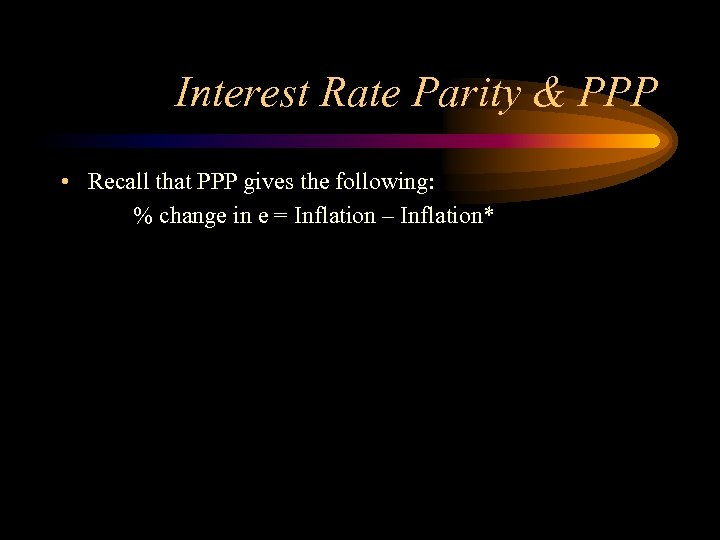Interest Rate Parity & PPP • Recall that PPP gives the following: % change in e = Inflation – Inflation*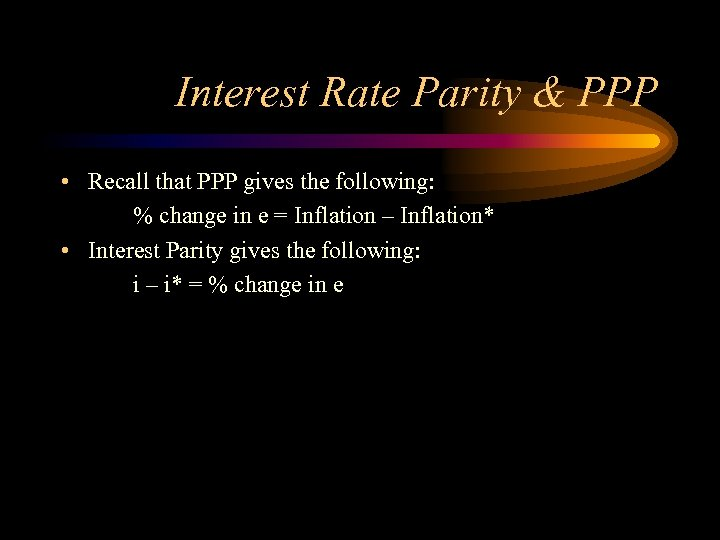Interest Rate Parity & PPP • Recall that PPP gives the following: % change in e = Inflation – Inflation* • Interest Parity gives the following: i – i* = % change in e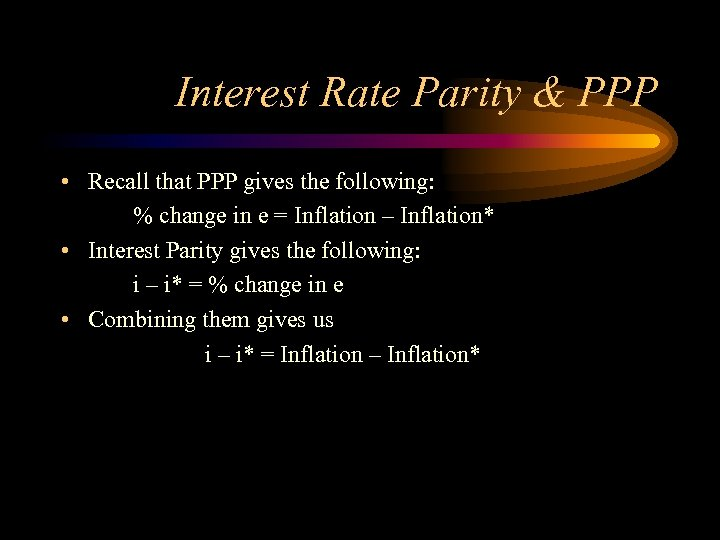Interest Rate Parity & PPP • Recall that PPP gives the following: % change in e = Inflation – Inflation* • Interest Parity gives the following: i – i* = % change in e • Combining them gives us i – i* = Inflation – Inflation*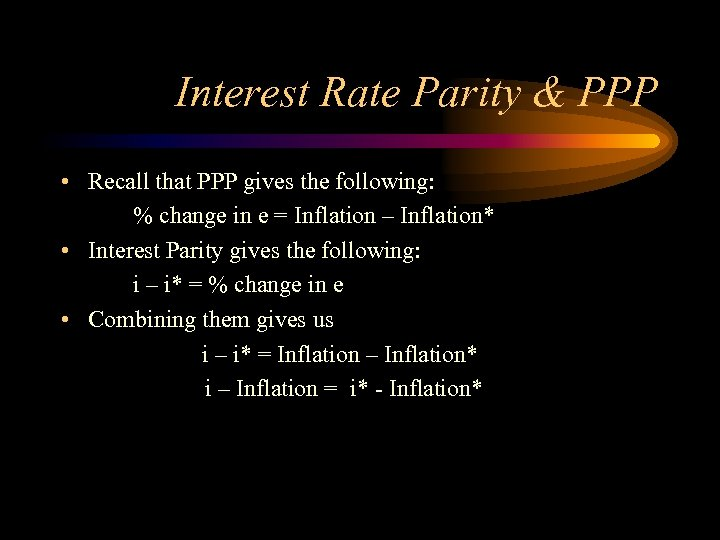Interest Rate Parity & PPP • Recall that PPP gives the following: % change in e = Inflation – Inflation* • Interest Parity gives the following: i – i* = % change in e • Combining them gives us i – i* = Inflation – Inflation* i – Inflation = i* - Inflation*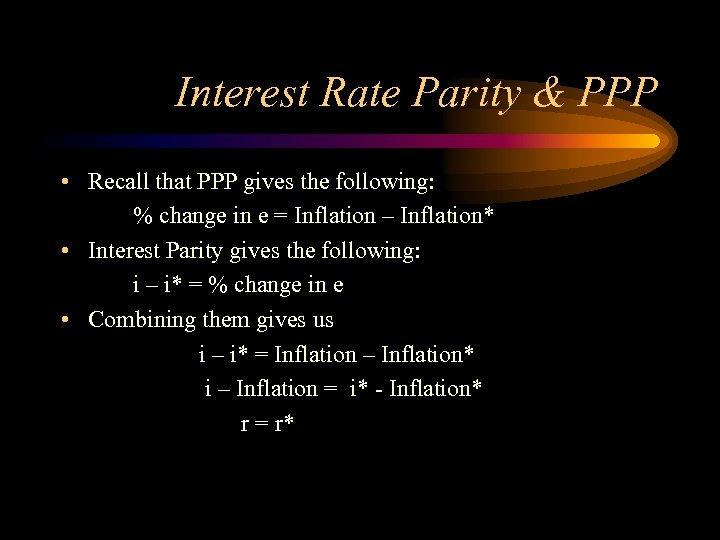Interest Rate Parity & PPP • Recall that PPP gives the following: % change in e = Inflation – Inflation* • Interest Parity gives the following: i – i* = % change in e • Combining them gives us i – i* = Inflation – Inflation* i – Inflation = i* - Inflation* r = r*